# 函数式编程

## Introduction to Functional Programming

Posted by R1NG on December 14, 2021 Viewed Times

# 函数式编程导论

1. 所有的函数 (Function) 视为 变量 处理: 允许 匿名函数 的声明, 允许将函数 作为其他函数的输入或输出.
2. 程序中的函数 视为 数学中函数 (映射): 其输出 只和它的输入有关, 如果两个函数的输入输出相同则 (在 Haskell 中) 它们 等价且可被彼此替换; 由此函数还不能执行任何意义上的 副作用.

## 1. Haskell 中的基本定义

### 对变量, 表达式和算式的基本定义方法

Haskell任何表达式和函数 都被统一视为 变量.

1
2
3
4
5
6
7
8
9
b = False || (False && True)

n = 1 + 7

b2 = n /= 8

b3 = (0,0) == (0,0)

v = 7 * (if b then 5 else 6)


### 对函数的基本定义方法

Haskell 中, 基本的 函数定义方法 包括 使用等式定义函数使用模式匹配 (实际上也就是不同的) 定义函数:

1
2
3
4
5
6
7
8
9
10
11
12
13
14
15
16
17
18
19
20
-- defining a function by an equation
add7 n = n + 7

-- defining a function by pattern matching
-- change the order and see what happens!

small 0 = True
small 1 = True
small n = False

-- defining a function on pairs by pattern matching
-- gives us more interresting examples

addUp (m,n) = m + n

-- we can also use _ to denote we don't care this input
first (e,_) = e


1
2
3
4
5
-- defining a reursive function

fib 0 = 0     -- base case
fib 1 = 1     -- base case
fib n = fib(n-2) + fib(n-1)     -- step case


### 对高阶函数的定义

1
2
3
4
5
6
7
8
-- this function takes a number and returns
-- the function which adds that number to
-- its input
addConst n m = n + m

-- we can test it by evaluating it at 3
-- and seeing what function we get


1
2
3
-- we can use anonymous functions to make it
-- obvious that this returns a function
addConstB n = \m -> n + m


1
2
3
-- indeed, we can define a function explicitly
-- using anonymous functions, rather than equations
addConstC = \n -> (\m -> n + m)


1
2
3
4
5
6
7
8
9
-- note: when a variable is used, it refers to
-- the definition closest  to the usage, so
-- the following returns the identity function, not
-- a constant function. This is called 'shadowing'
h n = (\n -> n)

-- but the following expression defines a function
-- which returns a constant function.
h2 n = (\m -> n)


1
2
3
4
5
-- we can define recursive higher-order functions
-- just like any other functions

repFromZero 0 f = 0
repFromZero n f = f(repFromZero (n-1) f)


### 其他有用的变量定义方式

#### case 语句

1
2
3
4
5
6
7
8
9
10
11
12
-- recall the definition of small above:
small 0 = True
small 1 = True
small n = False

-- we define a function which returns
-- True when it's argument plus one is small
-- using a case expression:
smallB n = case n + 1 of
0 -> True
1 -> True
n -> False


#### guard 语句

1
2
3
4
5
6
7
8
9
10
11
12
13
-- a guard expression lets you define a value
-- differently in different situations, defined
-- by boolean expressions.
-- It can also contain a 'where' clause to define
-- a repeated part of the boolean conditions.

sideOfFive n

| d > 0 = 1
| d < 0 = -1
| otherwise = 0    -- represent final/default case

where d = n - 5


#### let 关键字

1
2
3
4
5
6
7
8
9
10
11
12
13
14
15
16
17
18
19
20
-- a 'let' expression allows us to name
-- a value and use it in an expression
-- it is useful for making long formulas

y = let x = 10 + 10     -- just a local definition of x
in x + x

-- the let expression also **exhibits shadowing**:
-- the innermost let below is the one used for the
-- meaning of x.
-- Note that the below is not an intruction to
-- 'change' x from 10 to 20. In Haskell nothing
-- ever changes! We are just saying that locally
-- we want x to be defined in various ways.

z = let x = 10
in
let x = 20
in x


1
2
3
4
5
6
7
8
9
10
11
12
13
-- now we can see that functions do behave just like
-- ordinary values in at least one way:
-- at the point the definition of f below, the local
-- definition of x is 5, so f is defined to be the
-- constant function 5.
-- We don't need to worry about local definitions
-- of x in other parts of the code to understand
-- what f does.

w = let x = 5 in
let f = \n -> x in
let x = 6 in
f 0


#### 其他的一些常见错误

1. 模式匹配中不定义默认情形:
1
2
3
4
5
-- not defined for all inputs
-- this causes a runtime error

oops True = True
--try running main = print (oops False)

2. 递归定义函数时构造用不终止的循环或不构造有效的 Base Case:

1
2
3
4
5
6
7
8
9
10
11
12
13
14
15
16
-- this recursive function is badly defined and will
-- run forever on inputs other than 0
eep 0 = 0
eep n = 1 + eep n

-- printing yikes will cause Haskell to run forever
-- searching for a number x such that x = x + 1
yikes = let x = 5 in
let x = x + 1

-- the following will also run forever:
-- even though there are plenty of values equal
-- to themselves, the defintion gives us no clue
-- about which one the programmer wanted

yikesB = let x = x in x


### 相关题目解析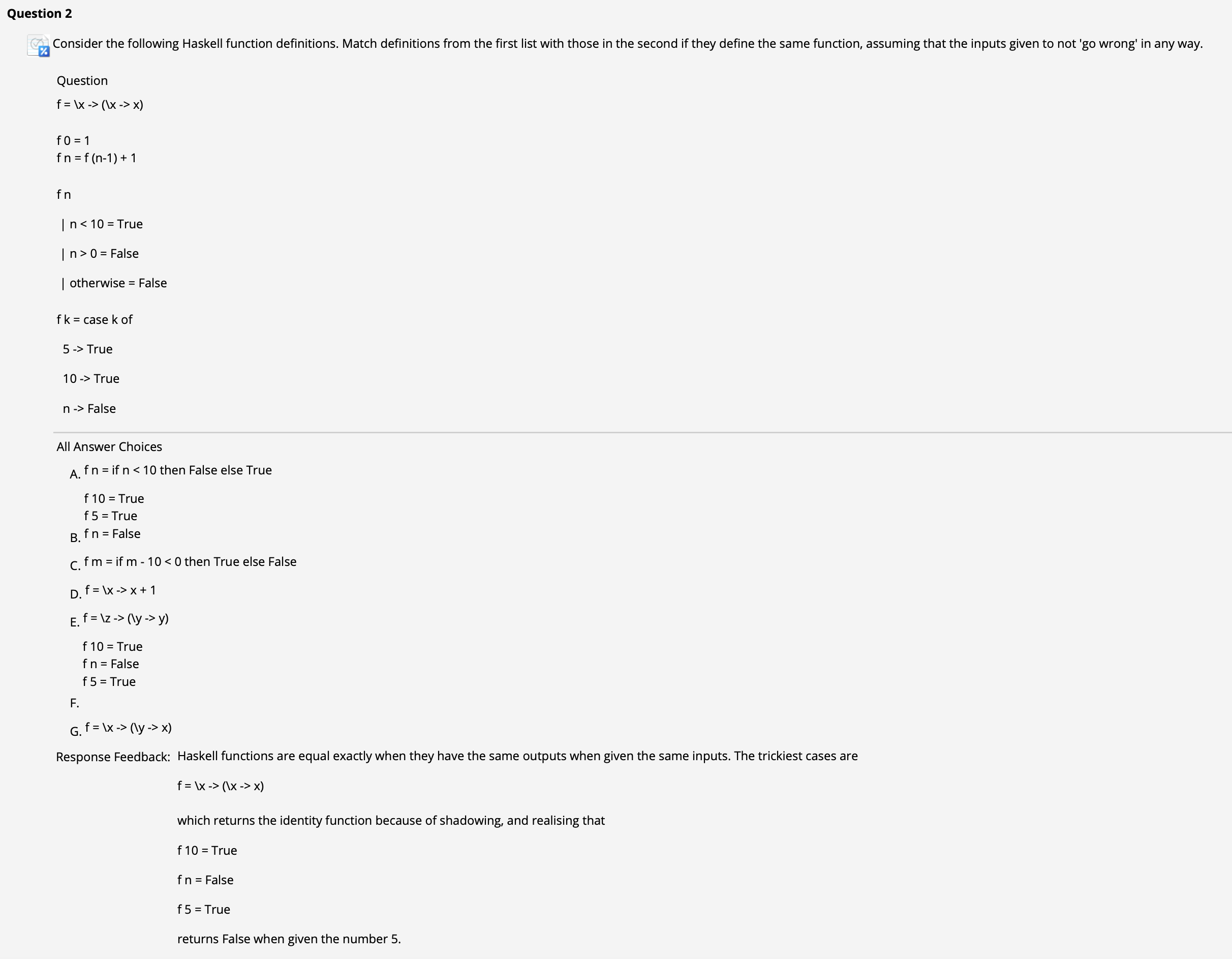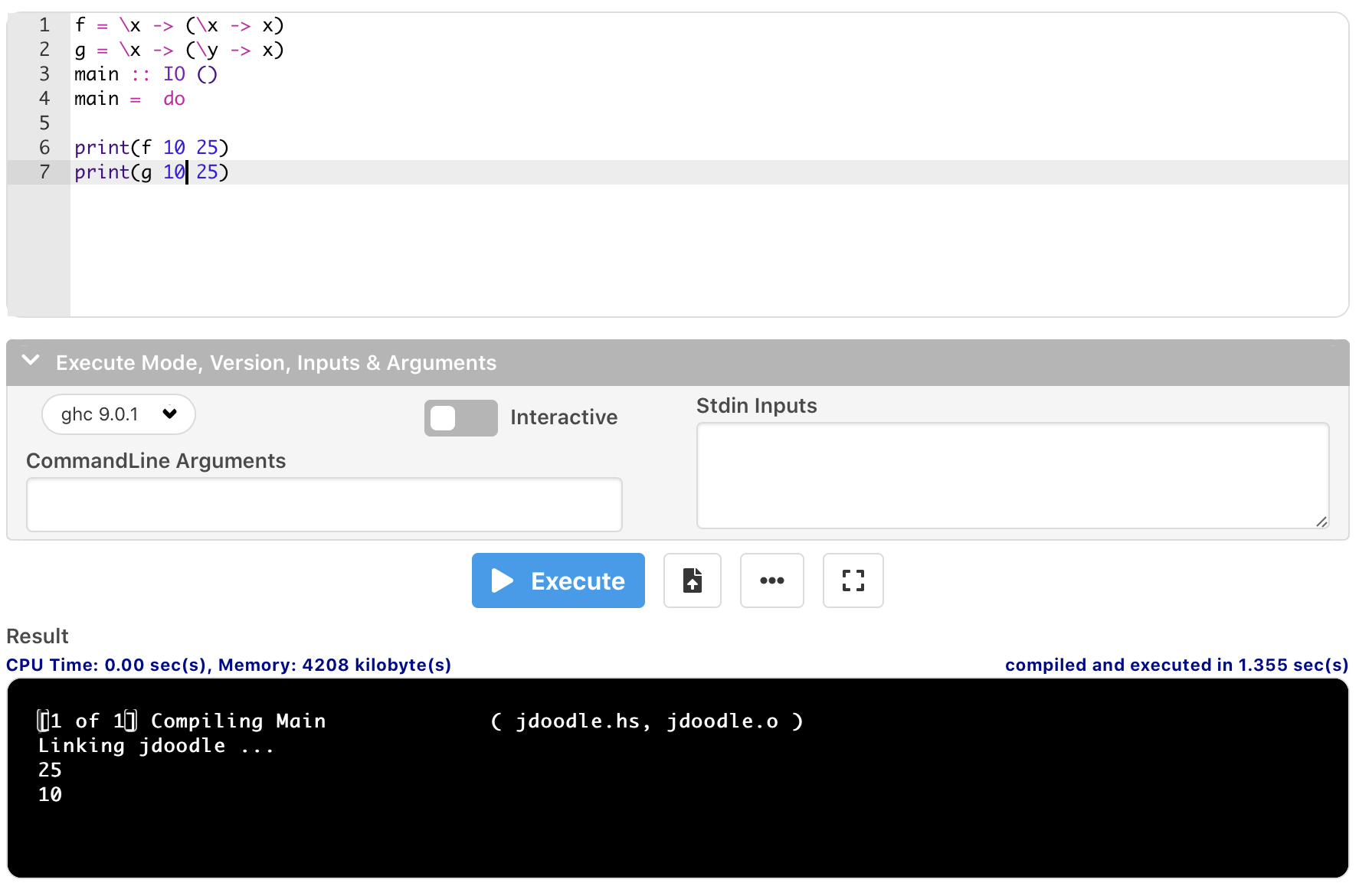## 2. Haskell: 类型系统

Haskell 中, 任何表达式均具备类型, 且对类型的检查发生在 编译阶段.

### Haskell 中的类型限制和自动类型推断

1
2
3
4
5
6
7
8
-- we can explicitly give the type of a function
f :: Int -> Int
f x = x + 1

-- Note: Int is fixed precision, Integer unbounded
-- We also have Float, Double
fd :: Double -> Double
fd x = x + 1


1
2
3
4
5
6
7
8
9
10
11
12
13
14
15
16
17
18
19
20
-- In Haskell, every expression has a type
-- How come last week we didn't have to
-- delcare them? Haskell infers types
-- we don't give explicitly E.g.
j x = True

-- What is the type of j?
-- We can give it several types, like
j :: Bool -> Bool
j :: Int -> Bool

-- Note we can't give it type Bool -> Int!
-- But the original has a strange feature:
main = print(j 1 && j True)

-- Is this 'dynamic typing'? No it is called
-- 'parametic polymorphism'. We can use
-- type variables to indicate that something
-- works for all types!
j :: a -> Bool


### Haskell 中的类型构造器 (Type Constructor)

1
2
3
4
5
-- We can use type constructors to make
-- more complicated types out of other types!
-- e.g. the type of pairs
sumPair :: (Int, Int) -> Int
sumPair (x,y) = x + y


1
2
3
4
5
6
7
8
9
-- Similarly, given two types we can construct
-- the type of functions from one to the other
atTen :: (Int -> Bool) -> Bool
atTen f = f 10
main = print(atTen (\x -> True))

-- note this is an example of type inference!
-- we could write explicitly
main = print(atTen ((\x -> True):: Int -> Bool))


1
2
3
4
5
6
7
8
9
10
11
12
13
14
15
16
17
18
19
20
21
22
23
24
-- We can use more than one type variable to indicate
-- which inputs have to have matching types
myApp :: (a -> b) -> a -> b
myApp f x = f x
main = print(myApp (\x -> True) 10)

-- note that it would also be correct
-- to give myApp a less general type e.g.
myApp :: (Int -> Bool) -> Int -> Bool

-- which still lets the example work:
main = print(myApp (\x -> True) 10)

-- but also
-- myApp :: (a -> a) -> a -> a
-- which doesn't! But it works for e.g.
-- main = print(myApp (\x -> 11) 10)
-- where the types are all the same

-- Note that we can't have
-- myApp :: (a -> b) -> b -> a
-- because this doesn't match what happens
-- in the definition: we can't apply a funtion
-- of type (a -> b) to an input of type b!


### Haskell 中的代数数据类型 (Algebraic Datatypes)

1. 认为 “选择型数据类型” (如 A|B型, 表示 “AB”, 以及枚举) 对应 代数和 (Sum)
2. 认为 “组合型数据类型” (如 AB型, 表示 “AB”), 对应 代数积 (Product).

Haskell 允许我们使用 data 关键字构造 用户自定义数据类型.

1
2
3
4
5
-- ALGEBRAIC DATA TYPES
-- we can make our own types by using the 'data'
-- keyword. The simplest are enumerations, which

data SwitchState = On | Off


1
2
3
4
5
6
7
8
9
10
11
-- we can define functions by pattern matching
toggle On = Off
toggle Off = On

isOn On = True
isOn Off = False

-- main = print(isOn (toggle On))
-- Note we can't do main = print(toggle Off)
-- because we haven't told Haskell how to print
-- a value of this type. (How to print "on"?)


1
2
3
4
5
6
7
8
9
-- we can also attach data from existing types
data MyIntPair = IntPair Int Int

mySumPair (IntPair x y) = x + y
main = print(mySumPair(IntPair 3 6))

-- we can also ignore some fields of a constructor
myfst (IntPair x _) = x
main = print(myfst (IntPair 1 2))


1
2
3
4
5
6
7
8
9
10
11
12
13
14
15
-- we can combine the ideas of enums and data
-- fields
data BoolOrInt = Abool Bool | Anint Int

-- This is called an 'algebraic datatype'
intval :: BoolOrInt -> Int
intval (Abool True) = 1
intval (Abool False) = 0
intval (Anint x) = x

opposite :: BoolOrInt -> BoolOrInt
opposite (Abool True) = (Abool False)
opposite (Abool False)= (Abool True)
opposite (Anint x) = (Anint (-x))
main = print(intval(opposite (Abool False)))


1
2
3
4
5
6
7
-- Algebraic datatypes can be recursive. This
-- is useful for recursively defined data structures
data MyList a = Empty | Append a (MyList a)

myHead (Append x l) = Just x
main = print(myHead (Append 10 (Append 11 Empty)))


### Haskell 中的列表语法

1
2
3
4
5
6
-- define a function take an integer list as input type
myIntHead :: [Int] -> Maybe Int

main = print(myIntHead ([1, 2, 3])))


1
2
3
4
5
6
myHead :: [a] -> Maybe a

-- String is defined as a list containing chars


1
2
3
4
5
6
-- quick defining numeric list:
[1, 3..10] <=> [1, 3, 5, 7, 9]

-- construct new list containing data from two disjunct lists, combination:
l = [(w, n) | w <- ""Hi!, n <- [1..3]]
-- result is: [('H', 1), ('H', 2), ('H', 3), ('i', 1), .., ('!', 3)]


### 相关题目解析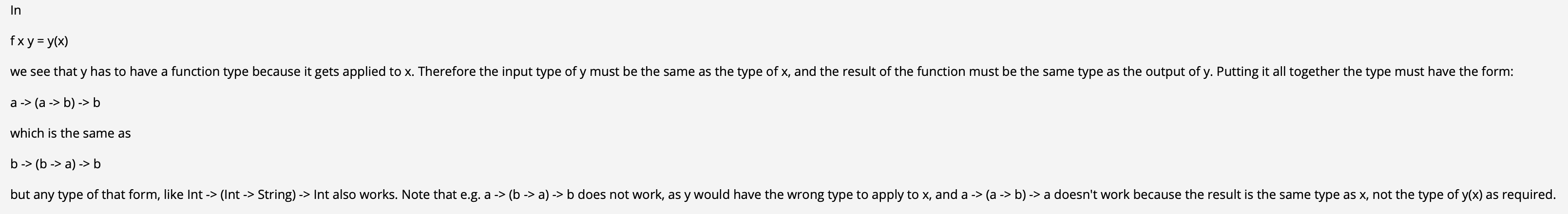## 3. 严格性

### Haskell 中的数值错误 Error Values

1
bottom = bottom


The term bottom refers to a computation which never completes successfully. That includes a computation that fails due to some kind of error, and a computation that just goes into an infinite loop (without returning any data).

1
2
3
4
5
eInt :: Int
eInt = error "Hang on..."

eBool :: Int
eBool = error "Hang on..."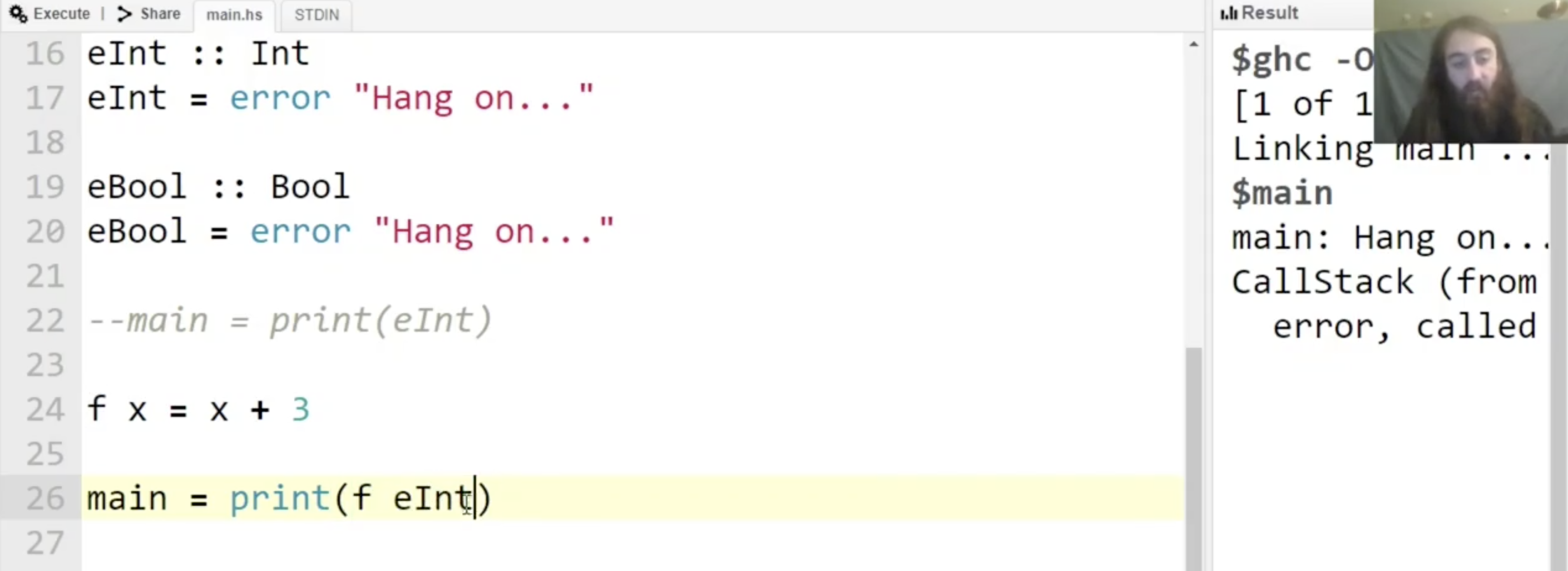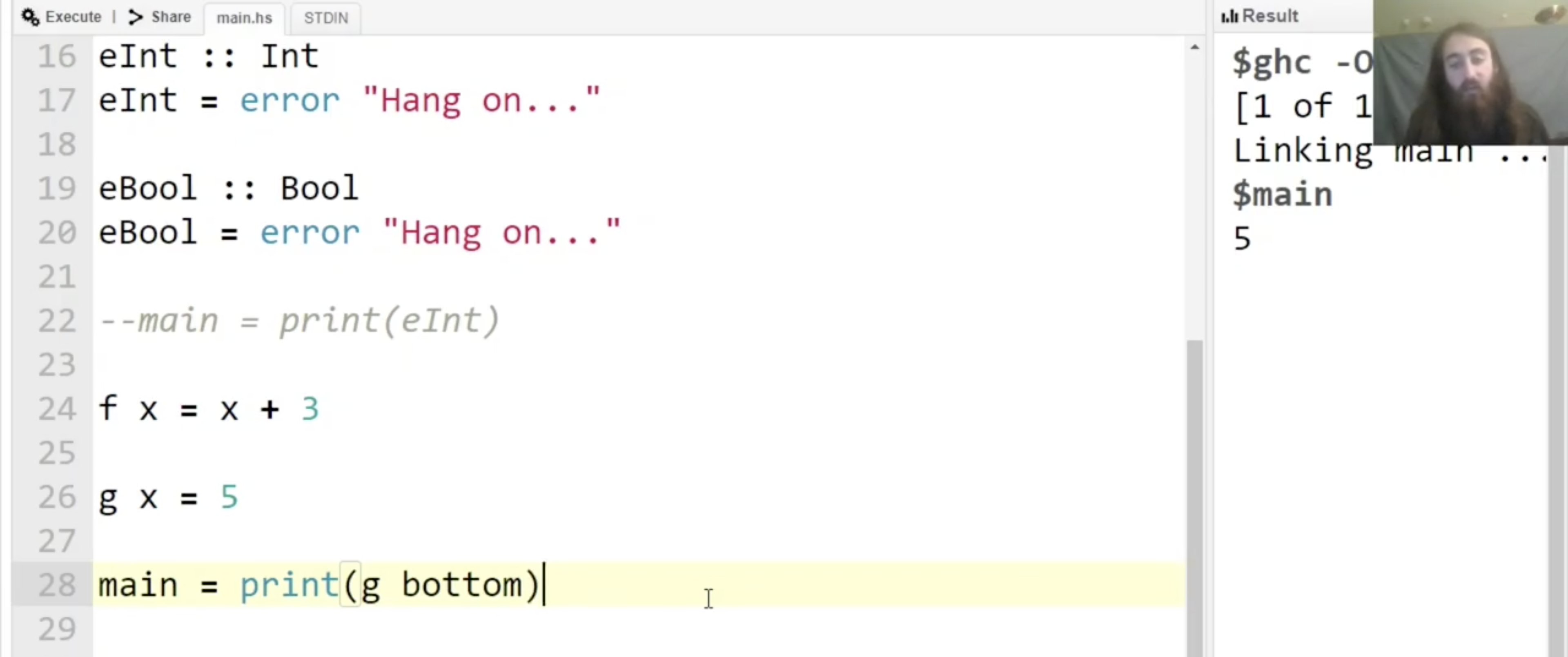### 在错误中推理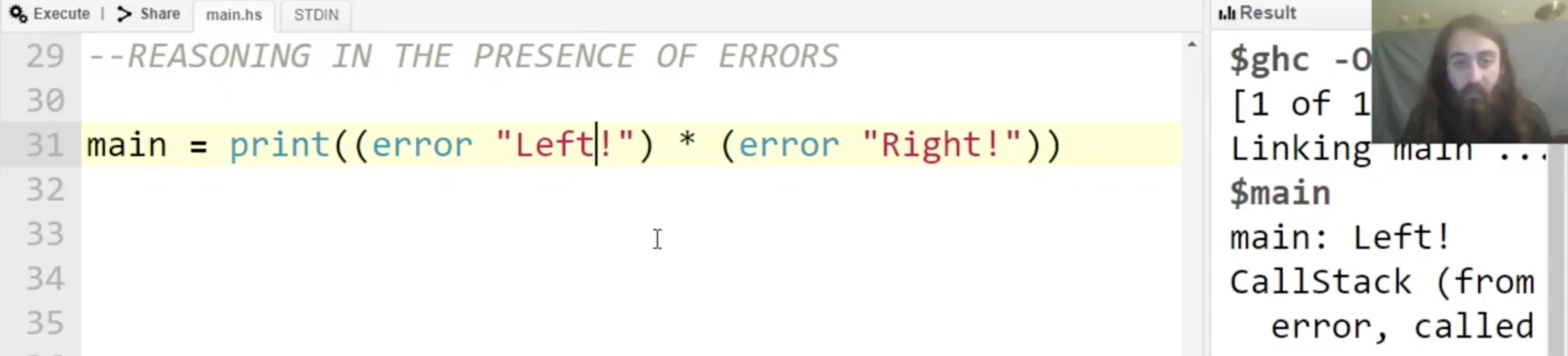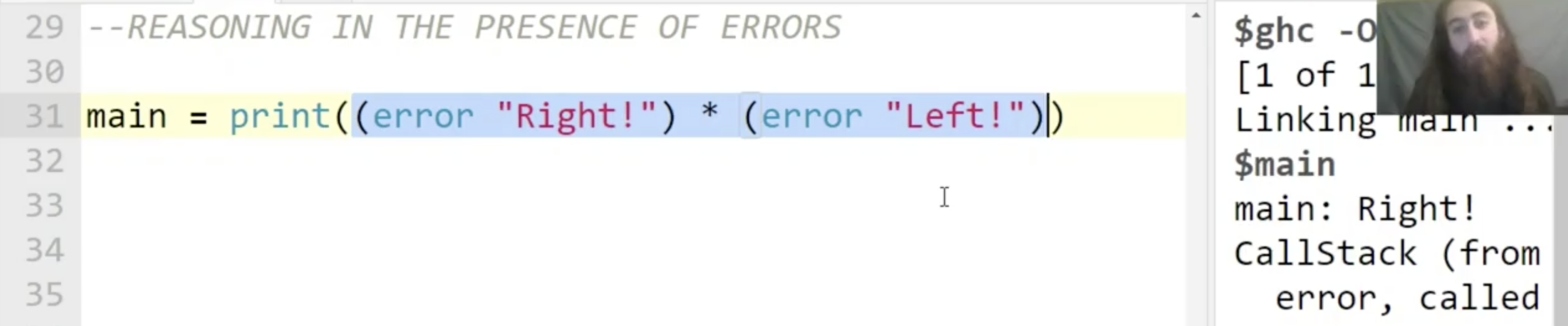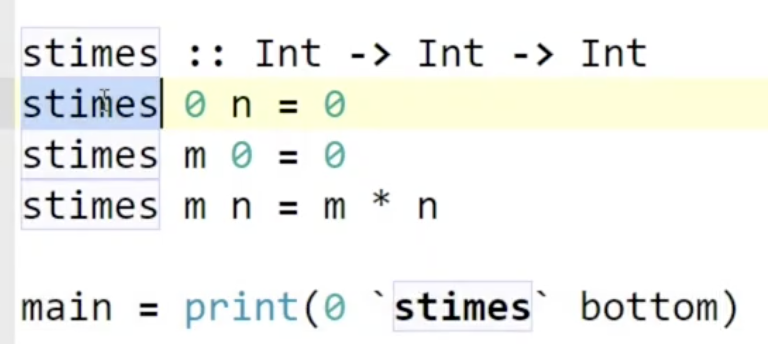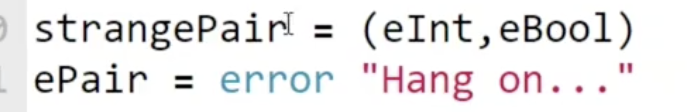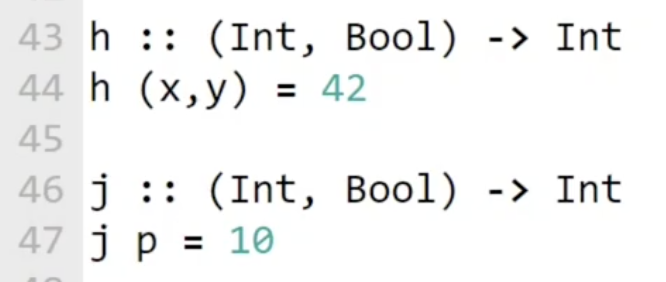Haskell 中, strangePair 被视为 包含两个 error 的二元组, 而 ePair 被视为 一个 error.

j 而言, 由于它对 参数的形状 没有限制, 因此对两种不同的输入它都能正常执行输出 $10$.

1
ePairb = (fst ePair, snd ePair)


Haskell 中的这一特性称为 对类型形状 (Spine) 的严格性 (Strictness).

### 无限的数据结构

1. mylen 对输入的 spine 不严格, 对输入的元素中的第一个也不严格, 但对输入的元素中剩下的部分的 spine 严格 (必须得是list).
2. mylen 并不会 evaluate 不需要被处理的元素, 也就是作为输入的列表里存储的任何元素.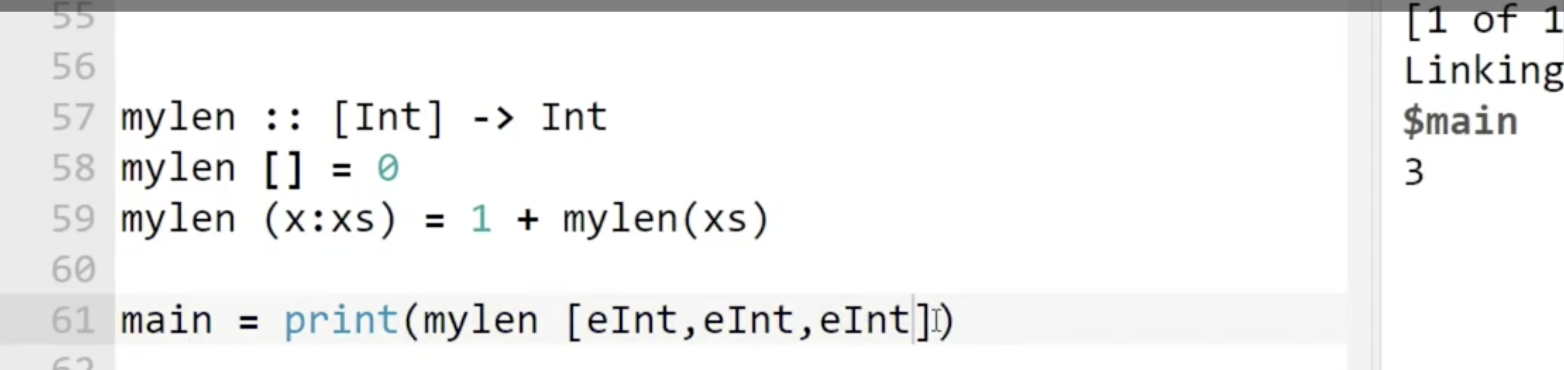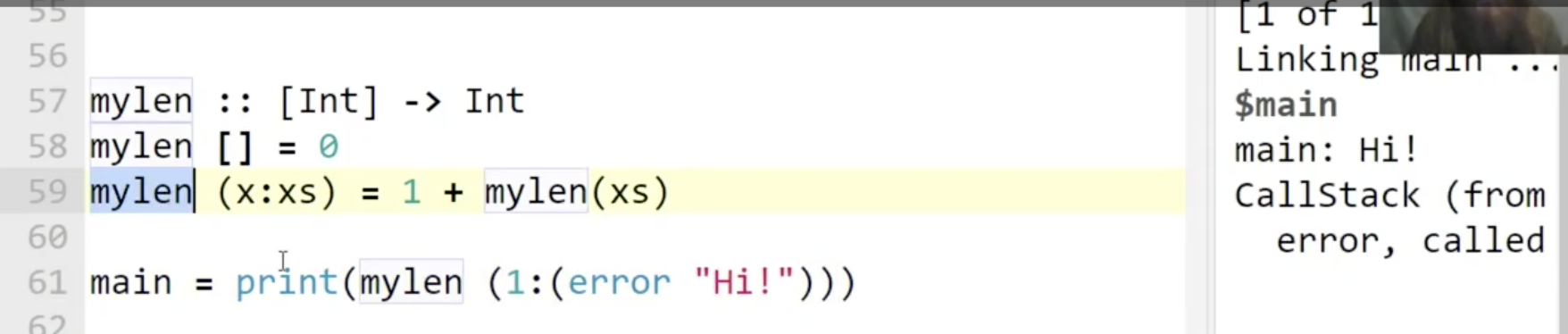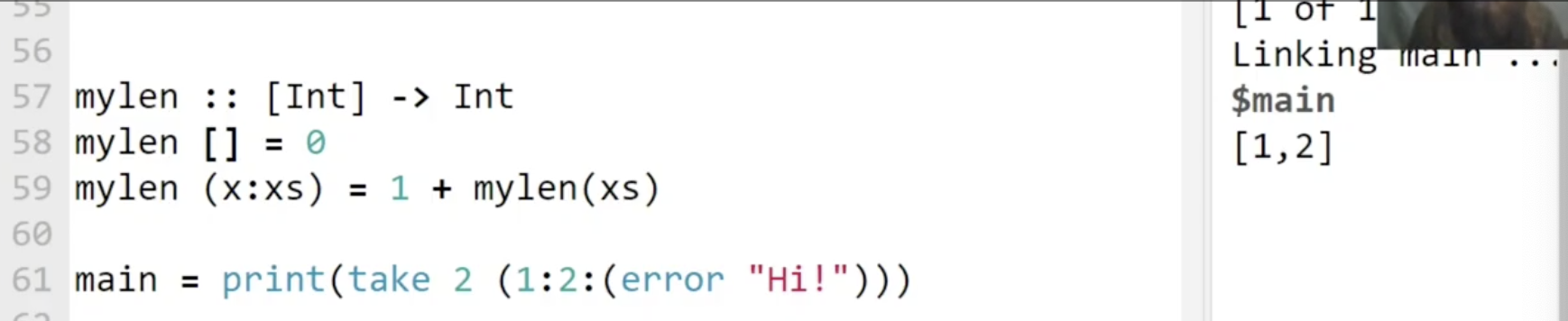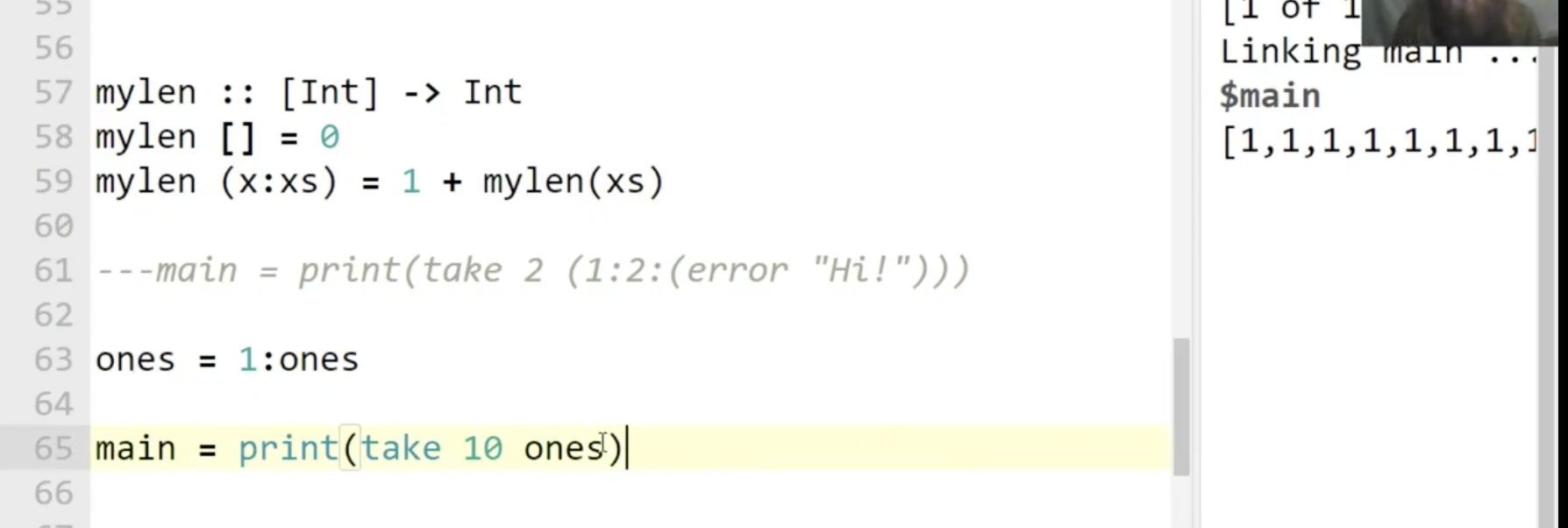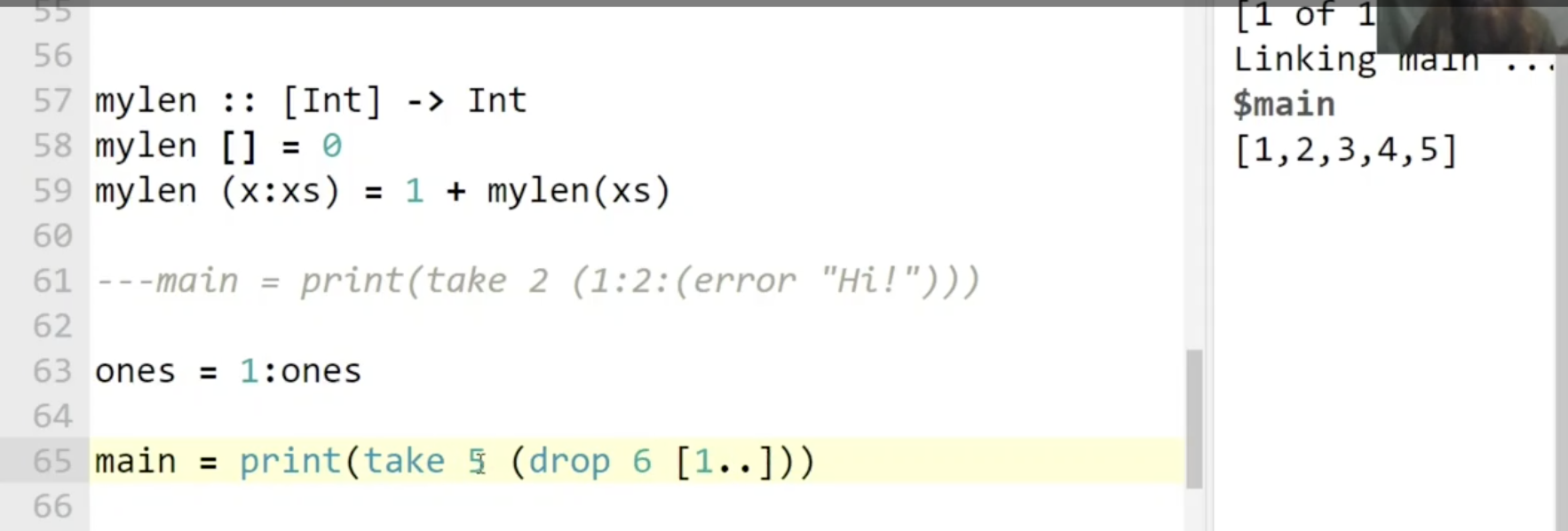1
nats = 0 : [n+1 | n <- nats]


Haskell 中, 内置算符 !! 用来对列表 indexing, list !! n 的结果就是从列表 list 中取出第 n+1 个元素, 因为列表编号从 $0$ 开始.

1
fibb = 1:1:[(fibb !! n) + (fibb !! (n+1)) | n <- [0..]]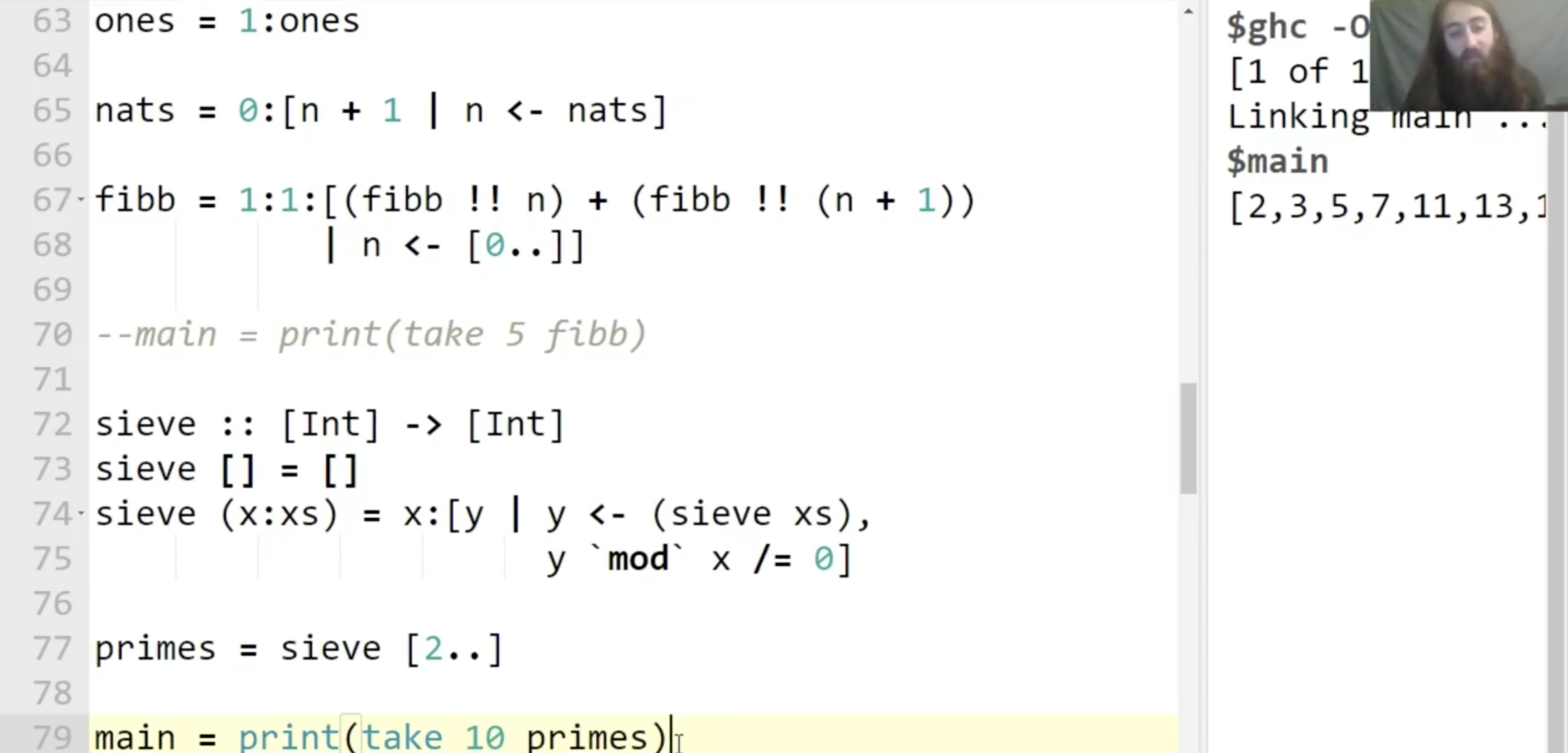### 相关习题解析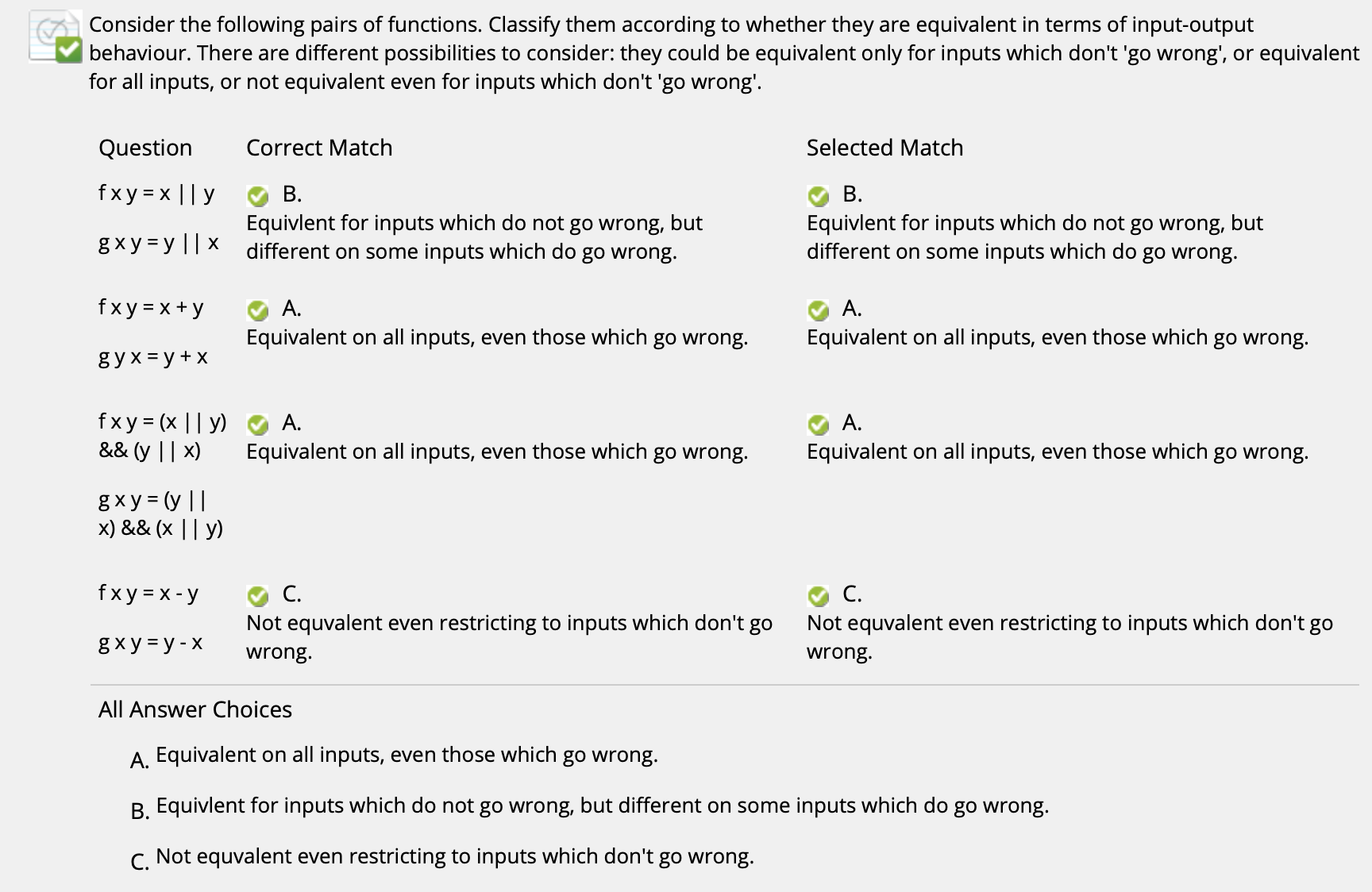($\uparrow$ 由于取并运算符 || 具有短路特性, 因此如果第一个元素为 $1$ 时它会返回 True, 但如果第一个元素是 error 的话就不一样了)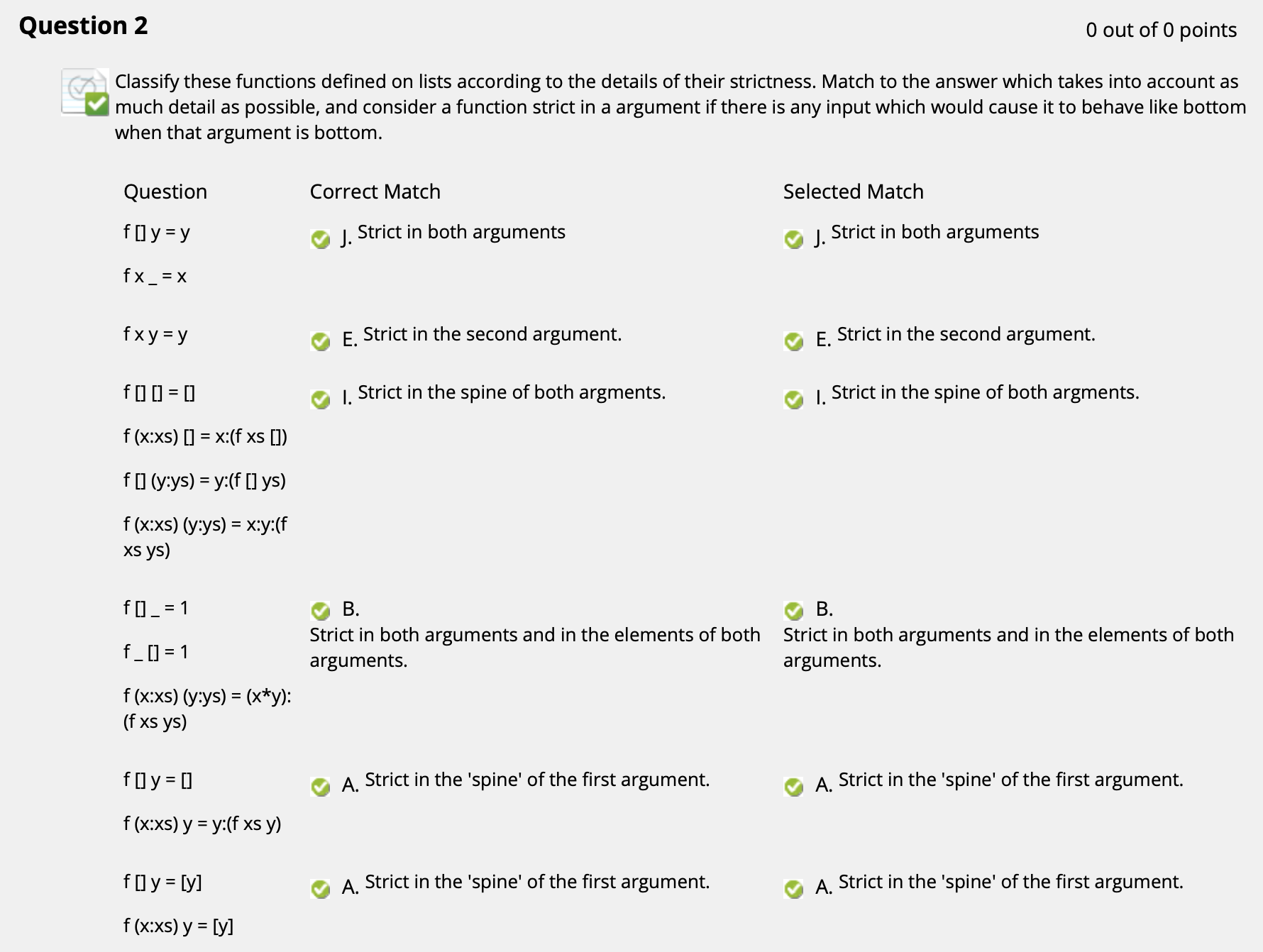($\uparrow$ 注意: 对第三个函数而言, 显然它在 base case 中就已经检查了全部两个变量的结构; 对第四个函数而言, 它不但检查了变量的结构, 由于存在 x*y, 乘法中任何变量都不能是 error, 因此它对于输入内部的任何变量也都是严格的. 对于最后两个函数而言, 它的输出不涉及对输入内部元素 y 的检查, 而是 原样输出, 因此函数对输入的内部元素 不是严格的.)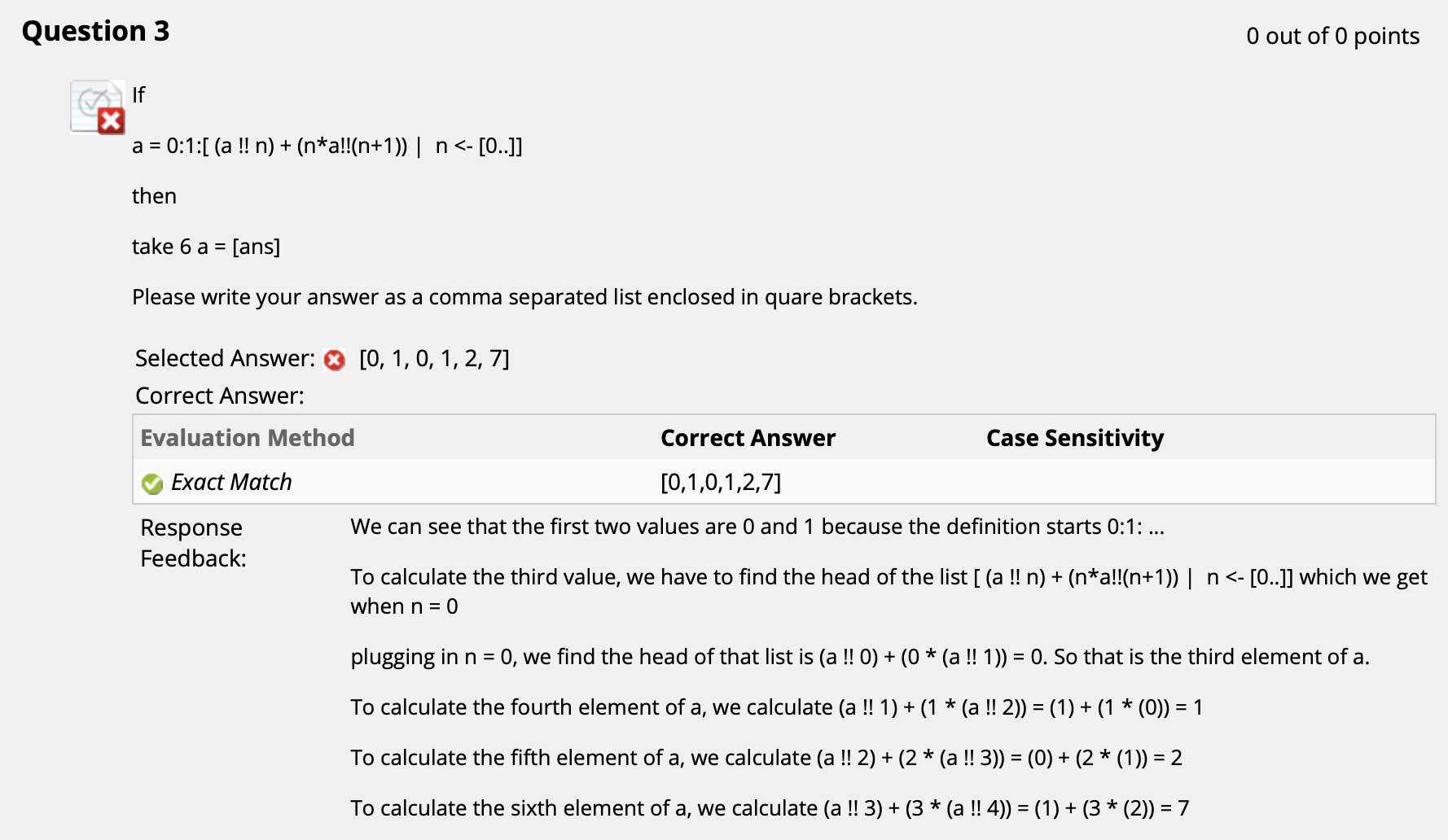($\uparrow$ 注意 !! 运算符是从列表中取对应位置的元素就可做出这题. 出错是因为 Blackboard 的类型匹配不太灵光…)

## 4. Haskell: 更多类型

### 参数 (Parametric) 多态和特定 (Ad-Hoc) 多态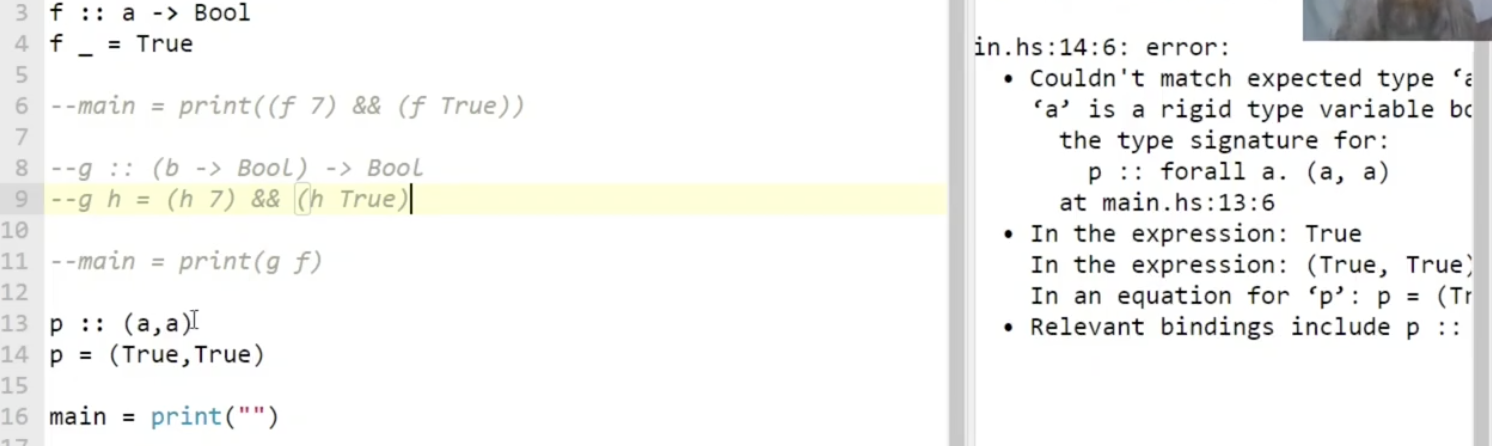Haskell 中最显然的特定多态例子就是 数值运算控制台输出. 我们使用类型不同的参数作为输入, 都可以对应地得到正确的结果.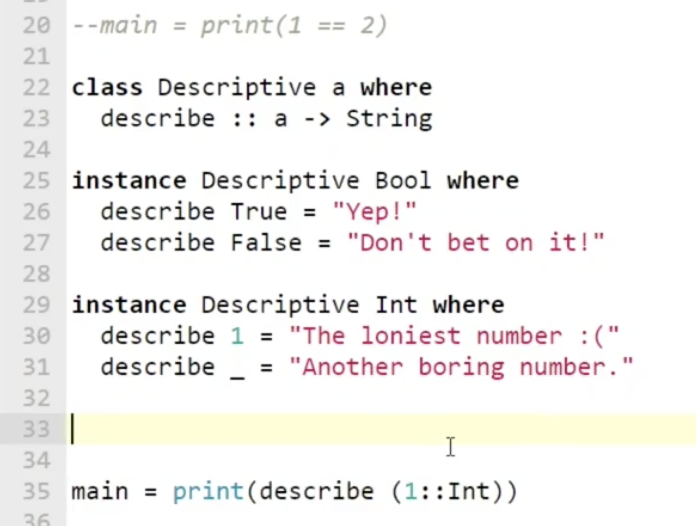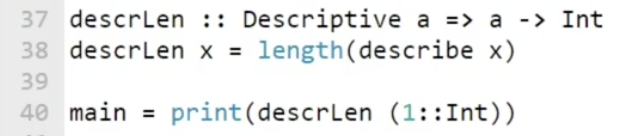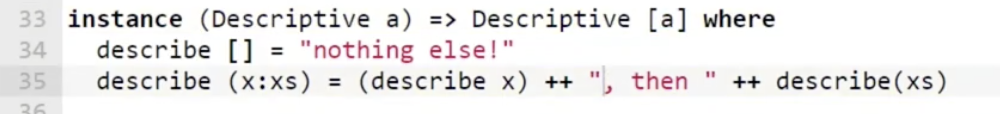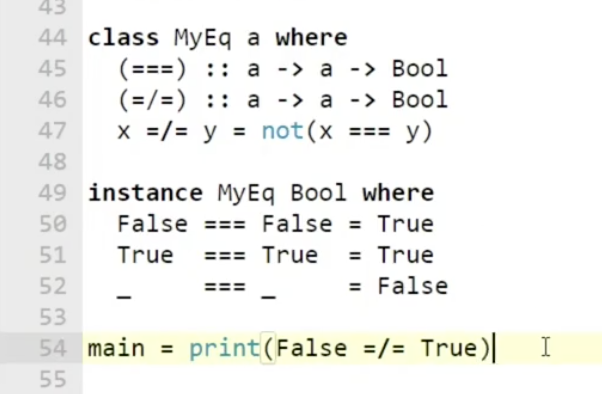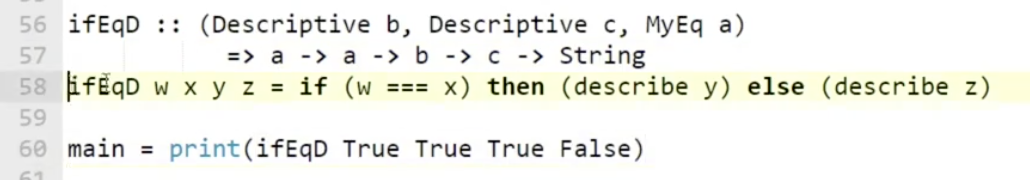Haskell 中, show 有一个默认实现, 但这个默认实现 不会被默认应用在用户自定义数据类型上. 考虑如下图所示的代数数据类型 PColor: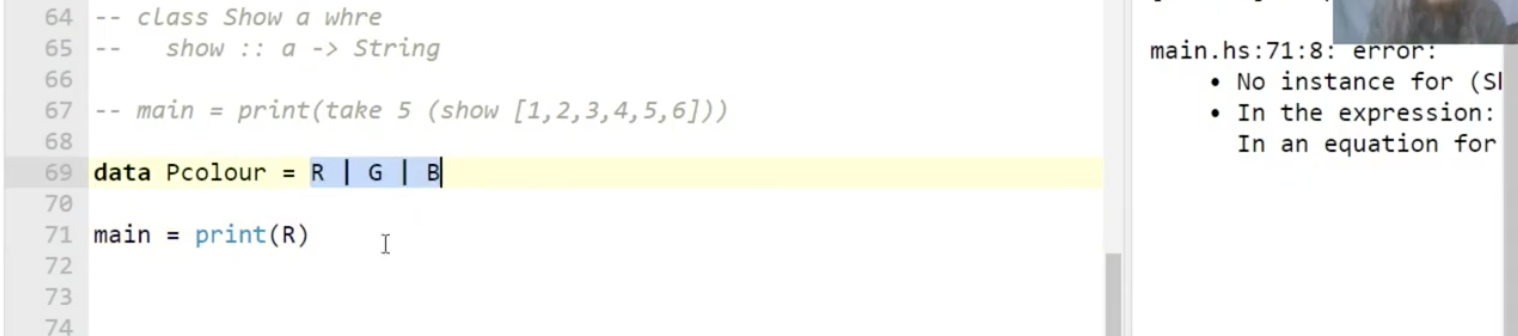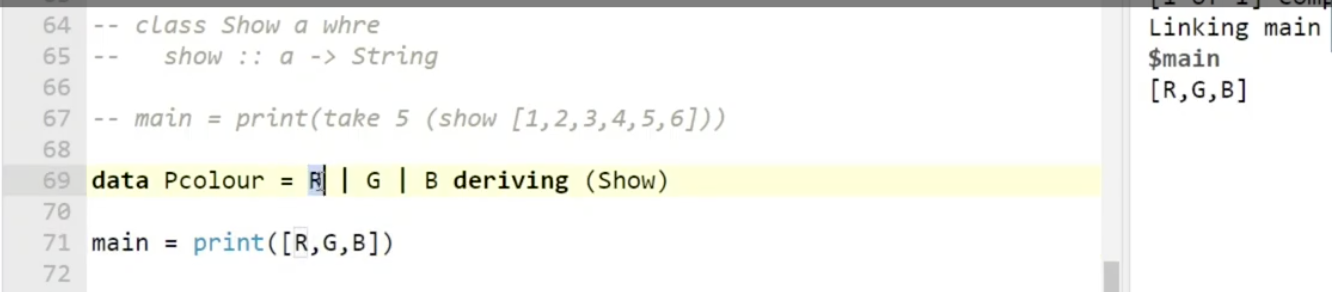Show 的默认实现之所以不会自动和用户自定义类型相关联, 是因为在正常情况下我们往往需要 自己实现对自定义数据类型的描述. 如下图所示: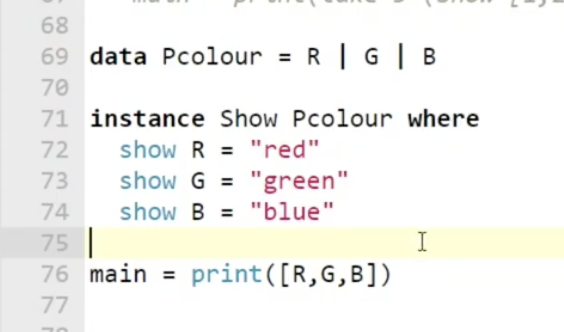### 相关题目解析: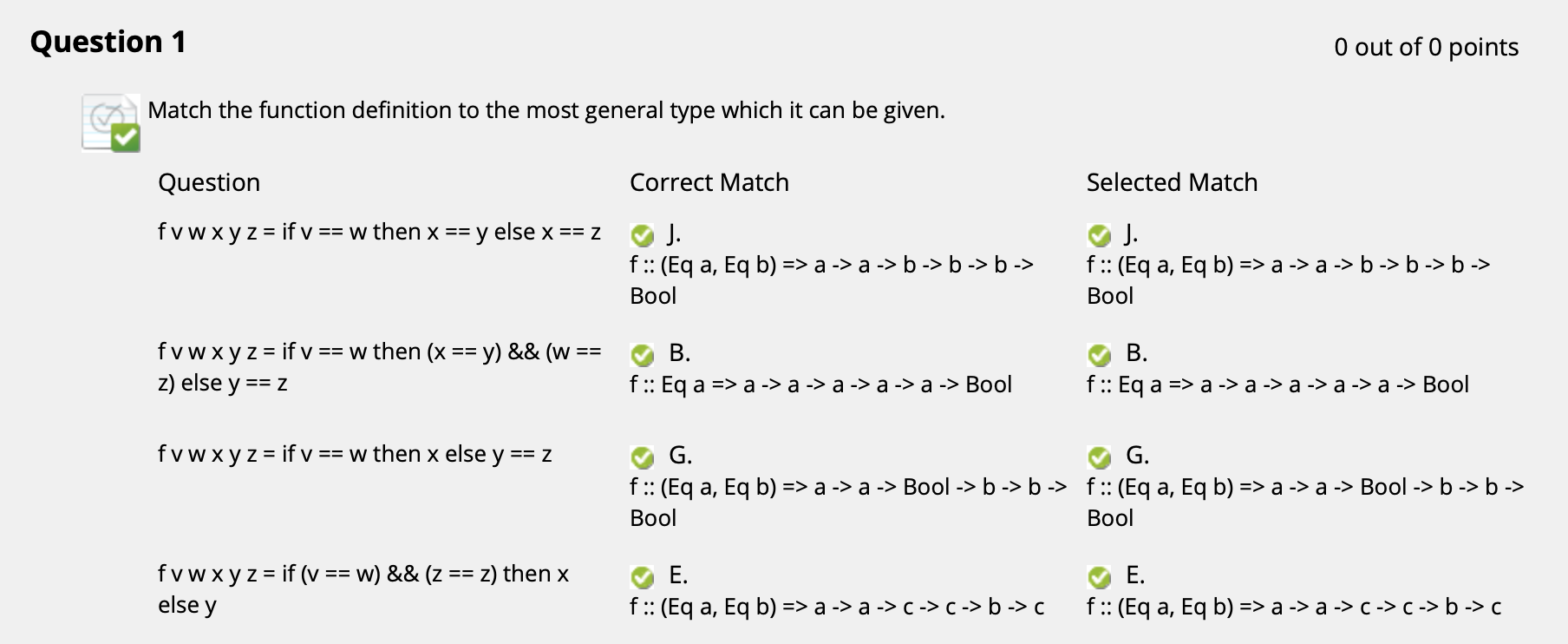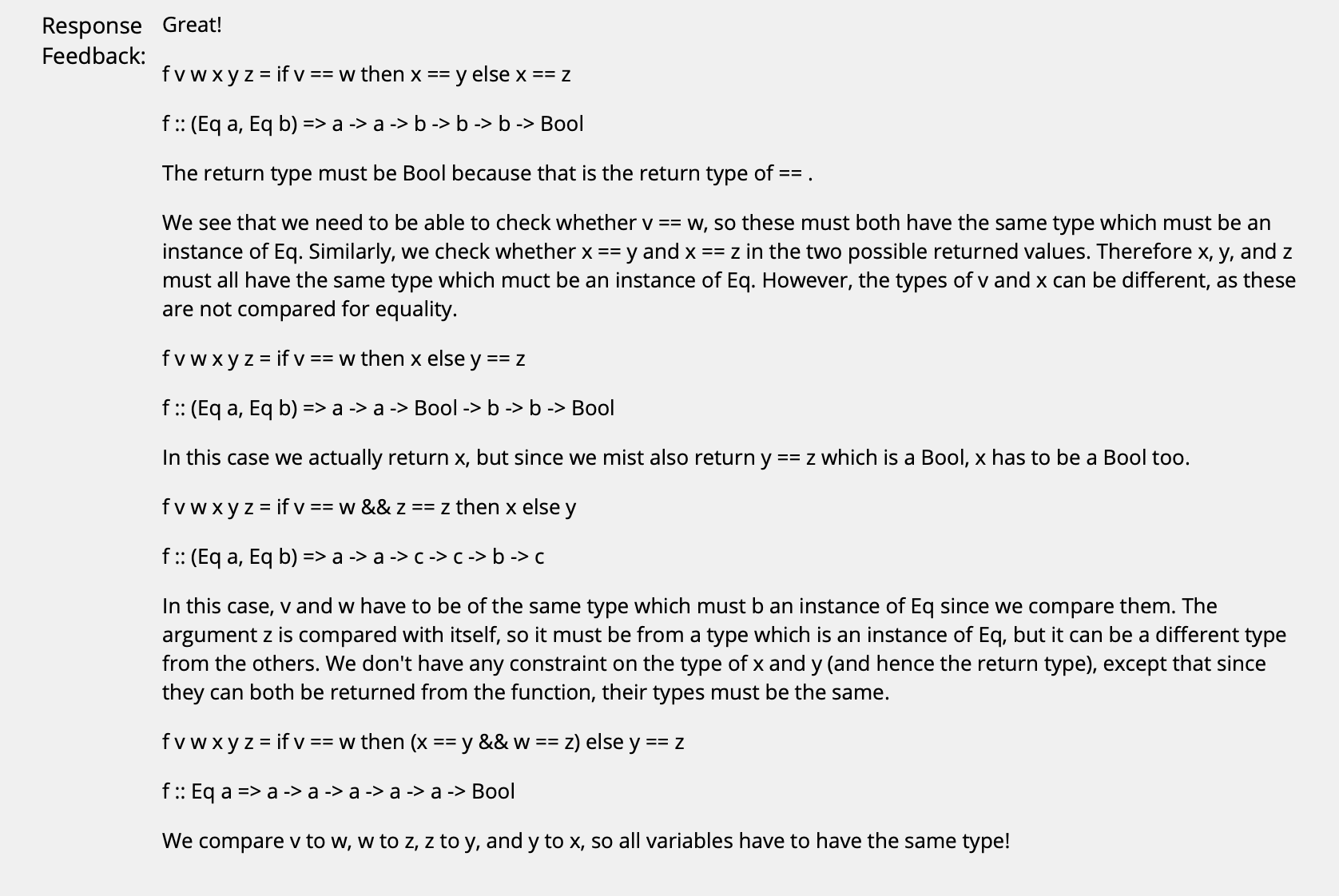($\uparrow$ 注意, 在涉及到 “==” (也就是 Eq) 时需要注意, 只有等式两边的变量类型相同才能比较是否相等. 如果有 Show 的话需要加上约束: 该变量被 Show 实现了, 也就是 Show x, x是对应变量. 我们还可以根据函数的返回值倒推变量的类型.)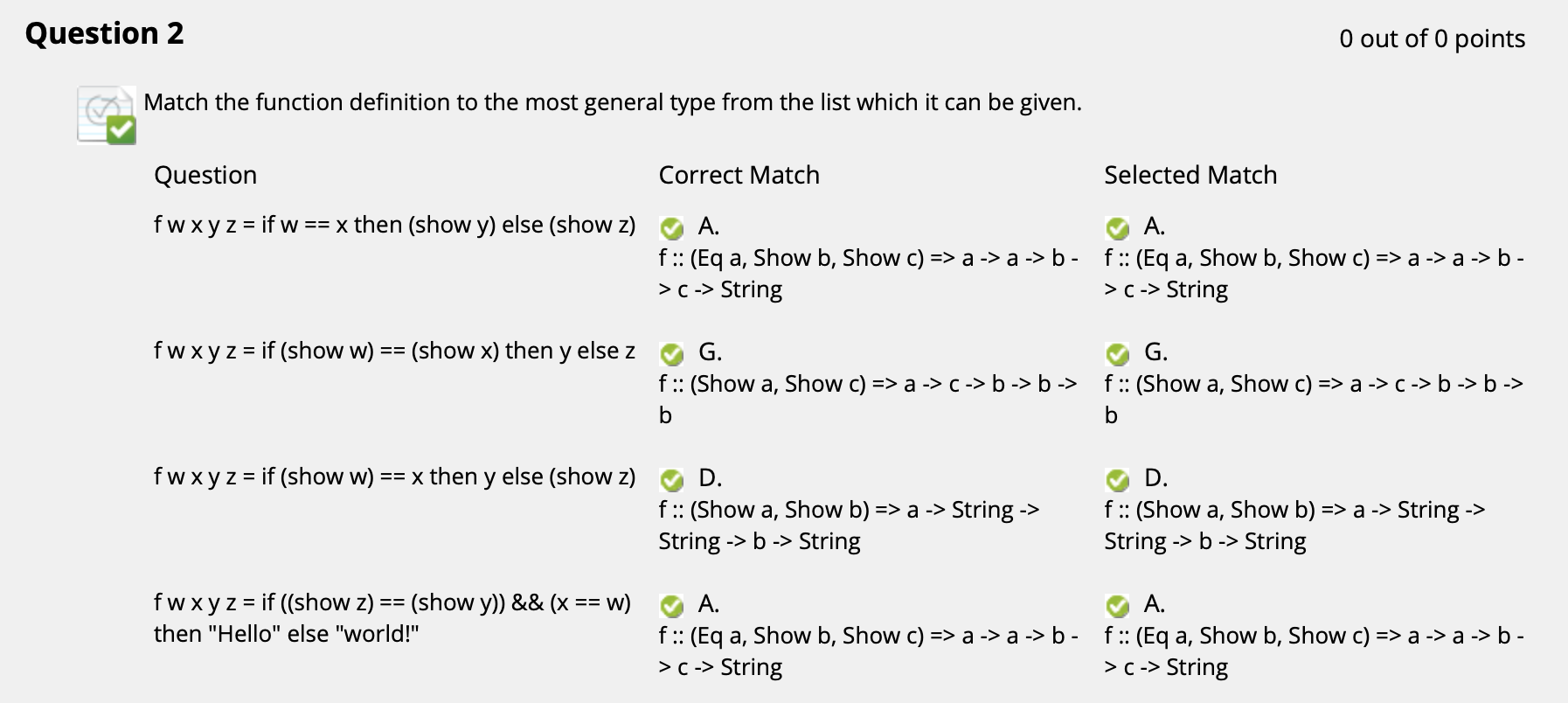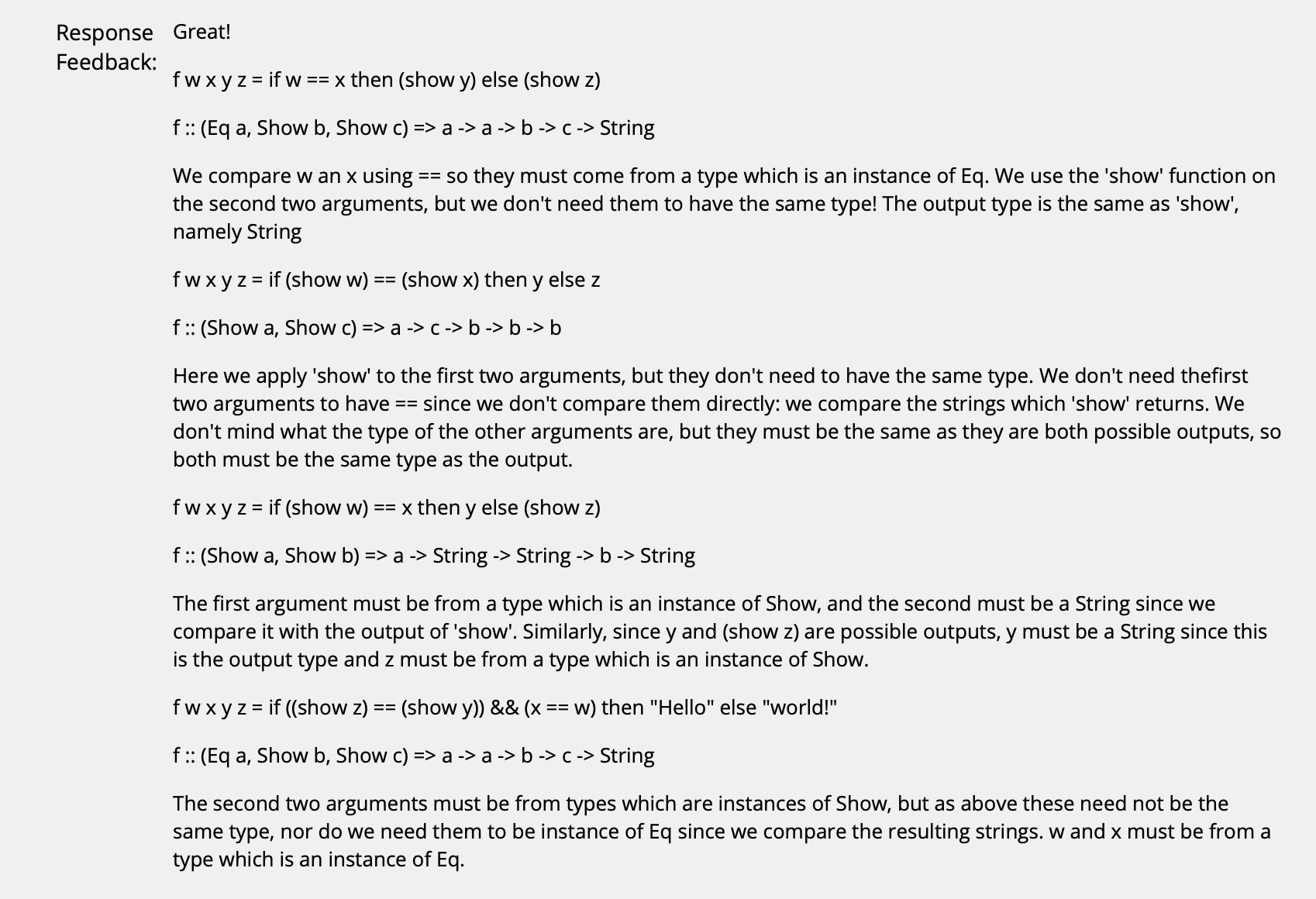($\uparrow$ 做这种类型的题时需要时刻注意: 一定要尽可能地选择最一般化, most general 的结果.)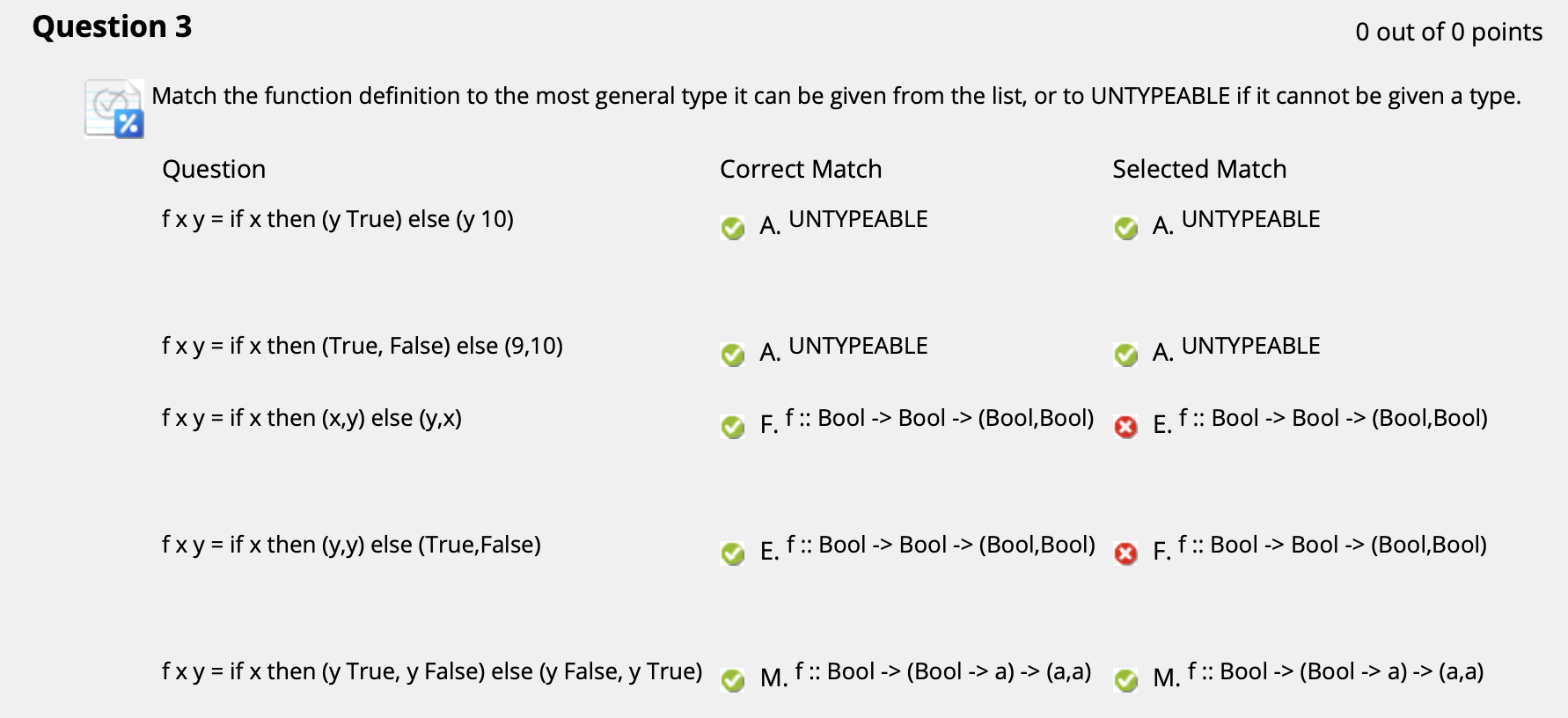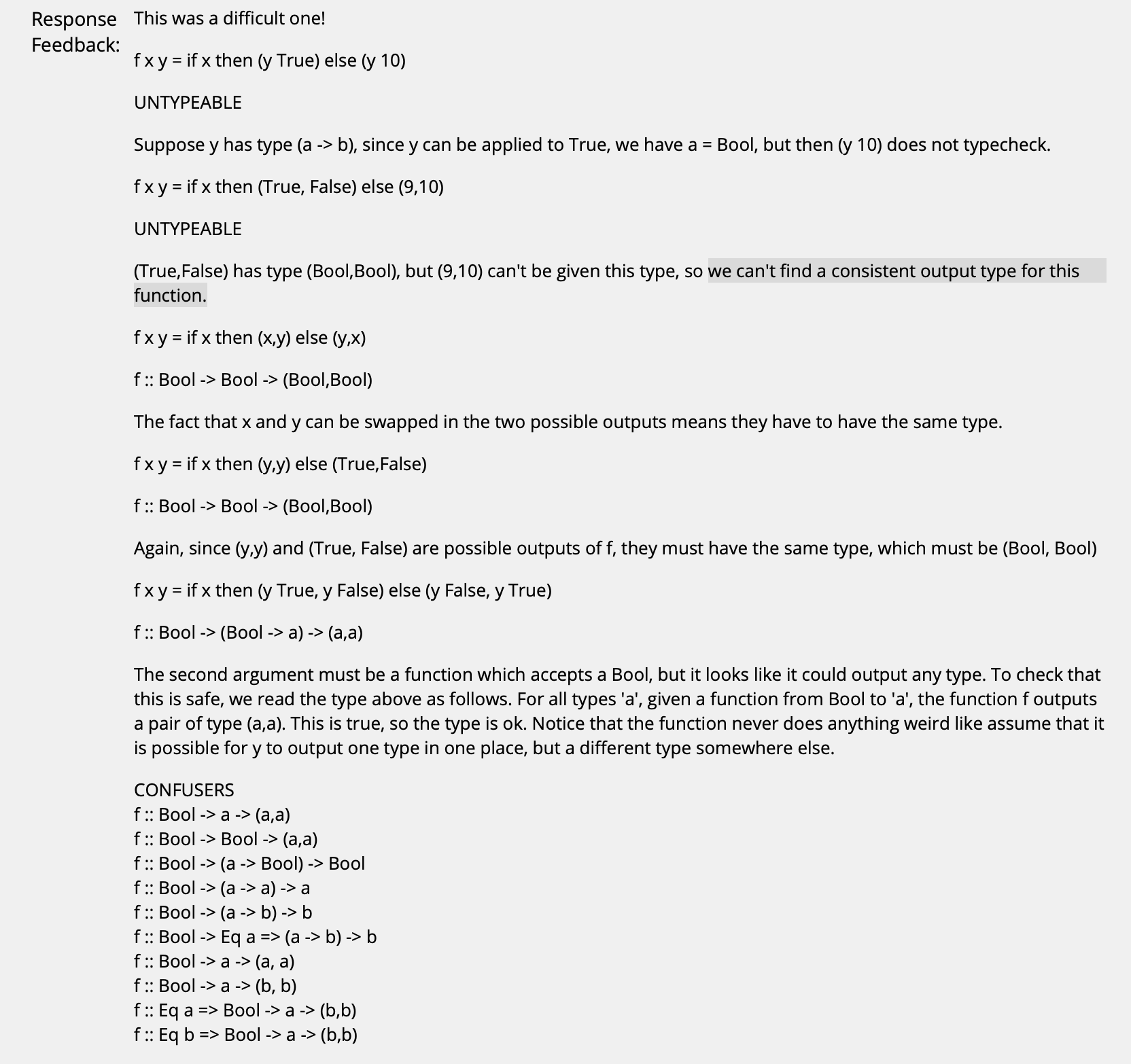($\uparrow$ 前两个是 untypable 的原因是, 它们的输出类型 不固定. 如果出现这种情况是无法赋予类型的! 即便我们指定函数的输出是某个 (b, b), 也不意味着类型 b 就可任意取值看人下菜, 实际情况下它始终有一个固定值!)

## 5. Haskell: 输入输出

Haskell 程序也可被视为一个 定义非常复杂的, 类型为 输入输出 (IO) 的变量. 而无论如何, 在任何 Haskell 程序中我们都 必须定义一个类型为 IO 的常量: main.

Haskell 编译器所做的事本质上可以视为: 它基于这个常量 main 的接收值计算它本身的值, 然后生成一个 具备对应输入/输出效果 的可执行文件, 仅此而已.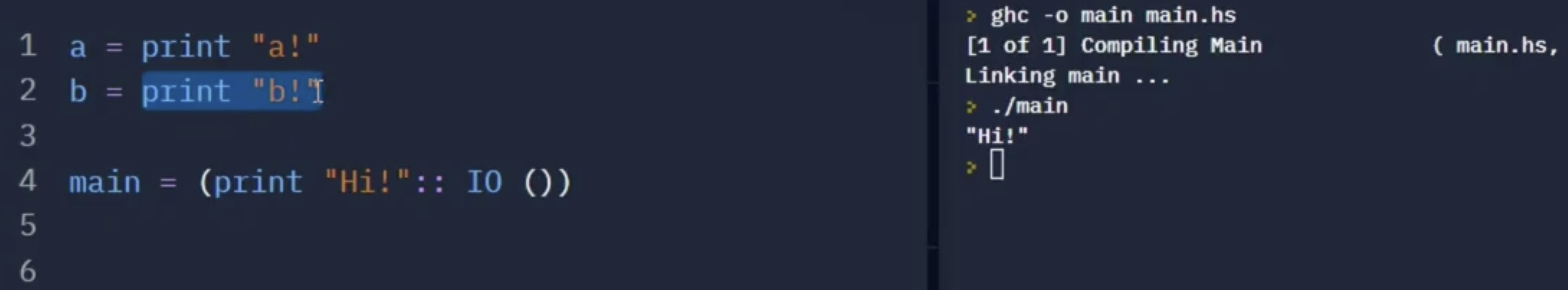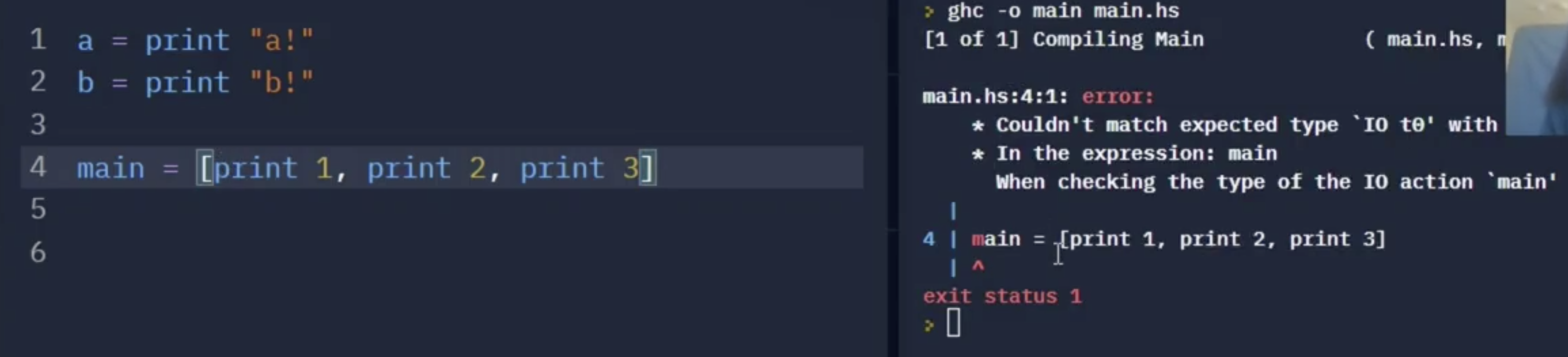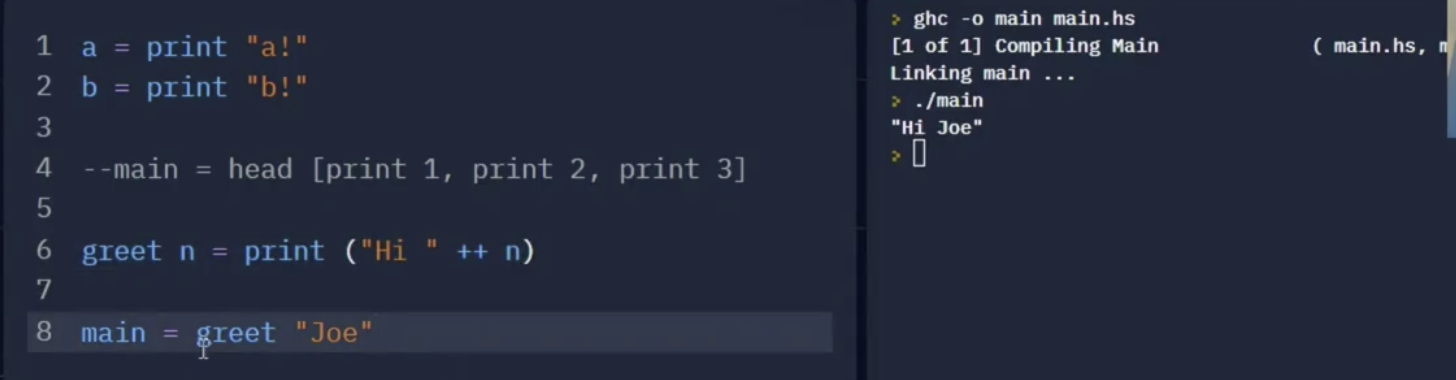return 不实际执行任何 I/O 操作, 但 “It make this variable being available to future IO actions that we might carry out”.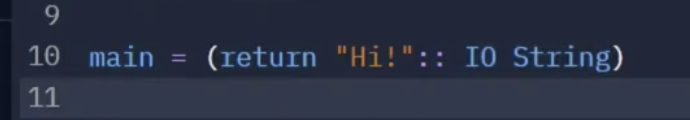I/O behaviour 的输入显然是 无法被确定 的. 因此, 它无法被作为 Haskell 中的常规数学变量, 以函数参数的形式直接参加运算.

Haskell 解决这一问题的方法是: 将需要对输入/输出变量进行的操作 (实际上也就是以输入/输出变量作为参数的函数) 嵌入到某个I/O 描述中:

Haskell 提供了一个用于 IO 变量 “feed” 到函数中的内置谓词: >>=:

1
>>= :: IO b -> (b -> IO c) -> IO c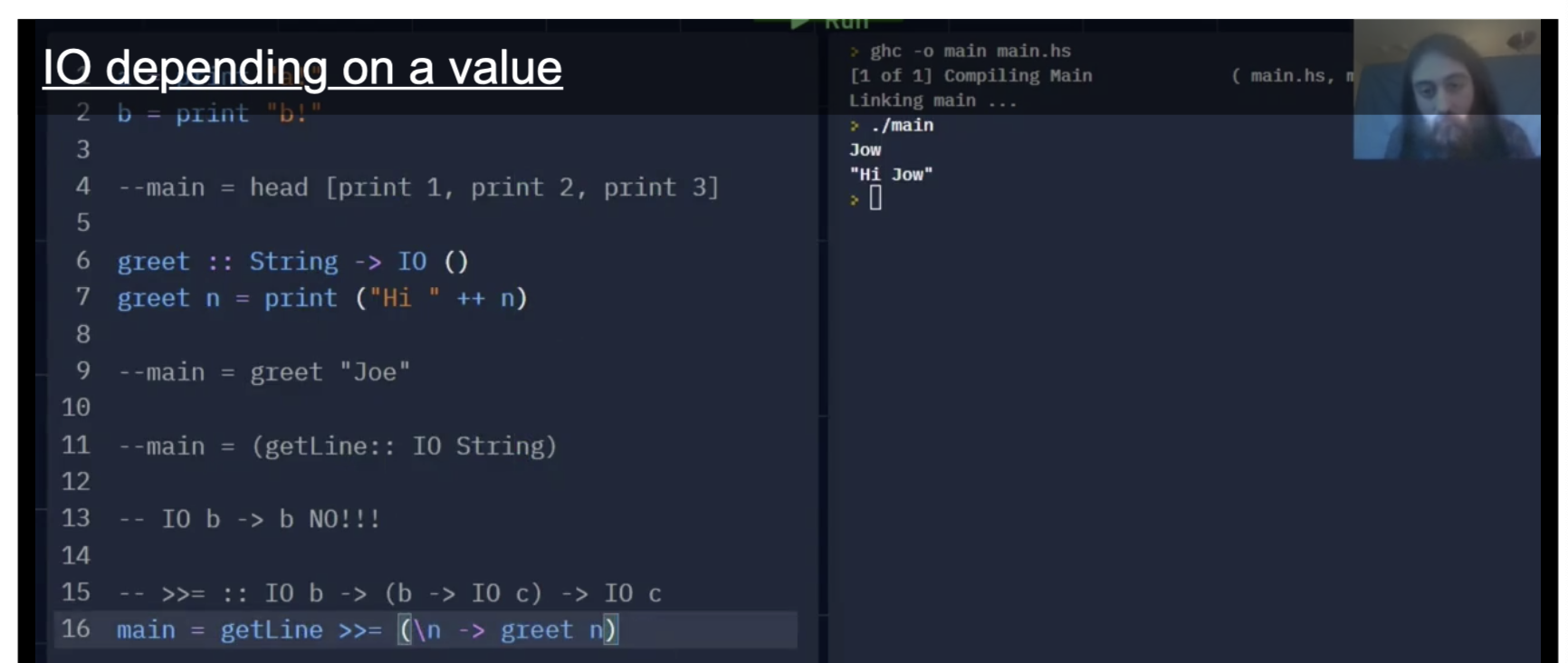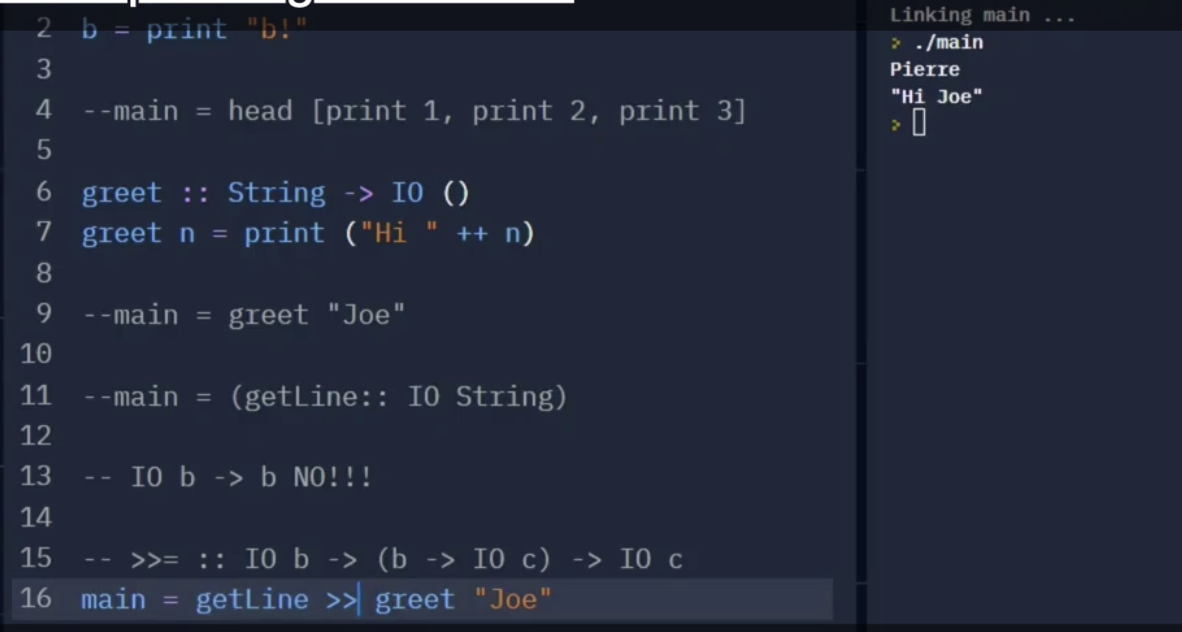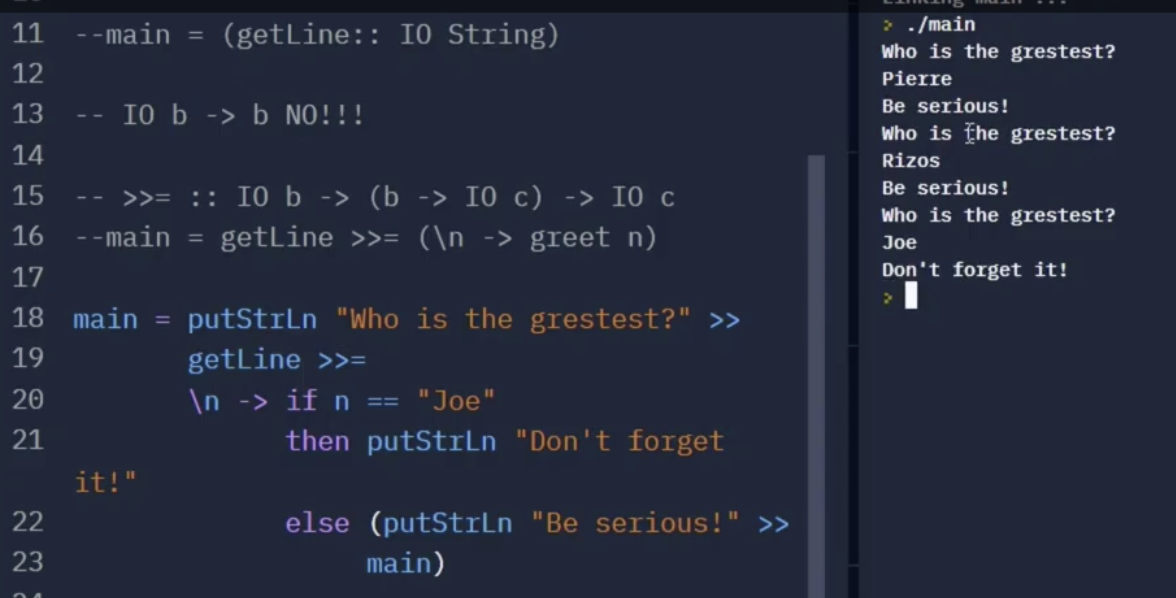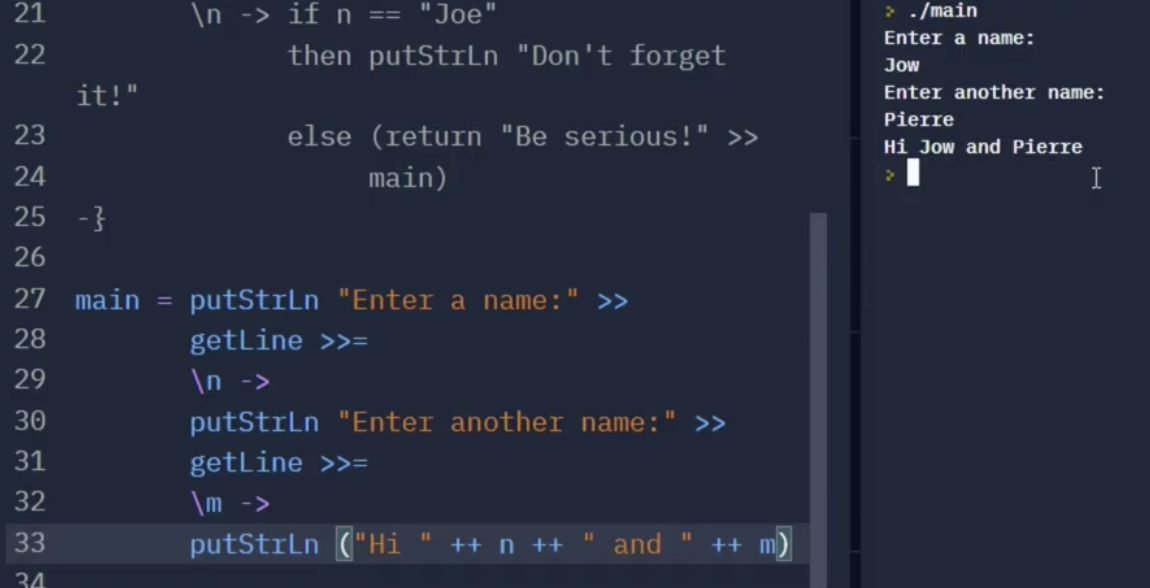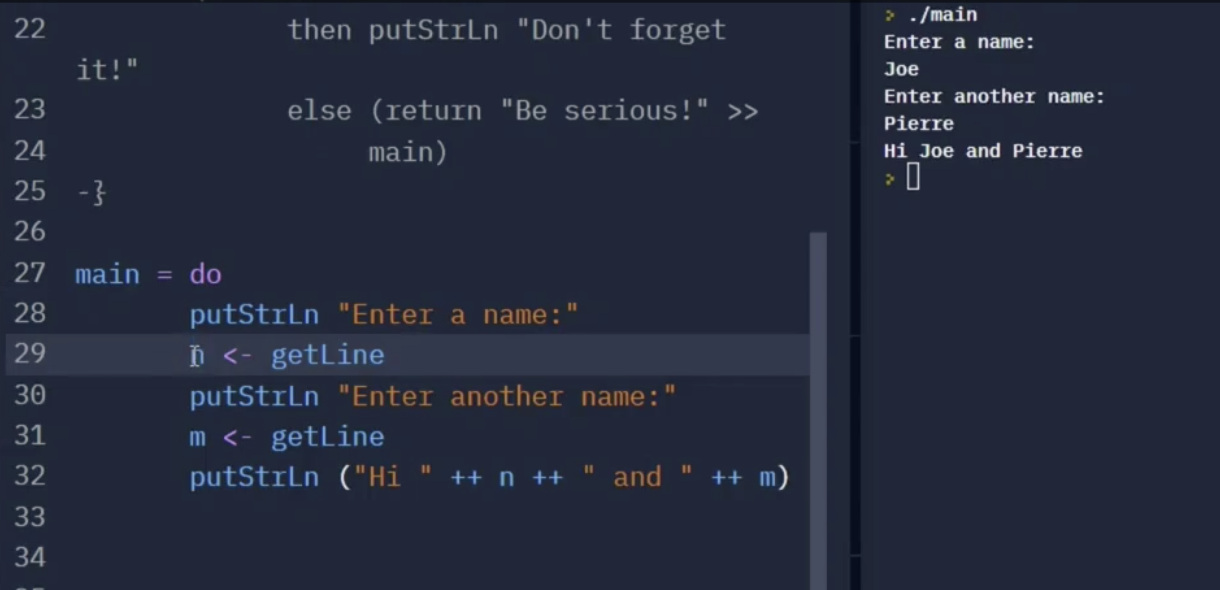### 相关题目解析: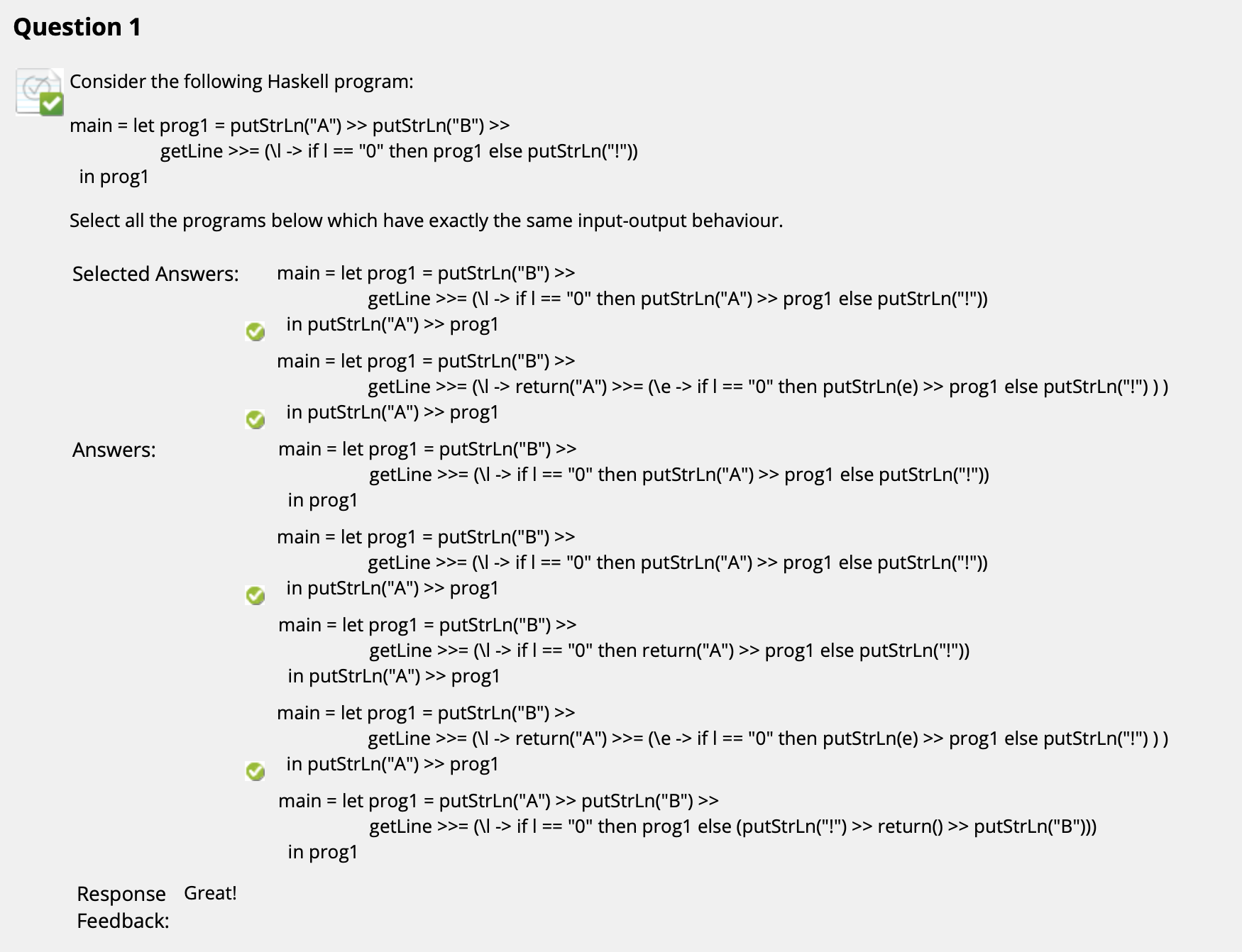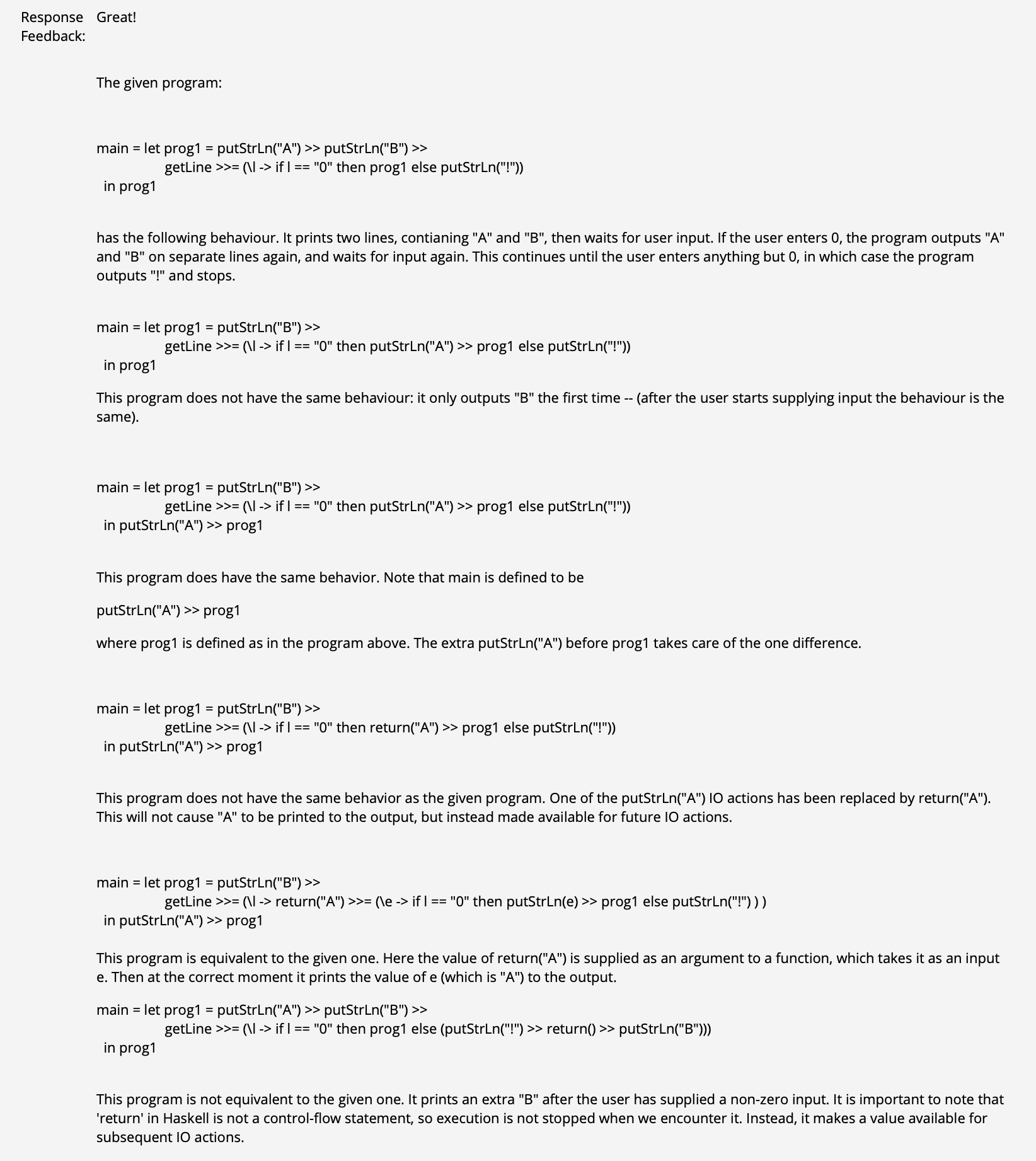($\uparrow$ 注意: 1. “return” 不产生任何实际的 IO 操作, 只是起到类似 “传值” 的作用. 2. 程序实际执行是从最底下 in prog1` 那一行开始的.)